• 基于Frenet坐标系的智能驾驶运动规划方法 Frenet坐标系基本概念及坐标系建立 FrenetFrenetFrenet 坐标系使用道路的中心线作为参考线，使用参考线的切线向量和法线向量建立坐标系，以车辆在参考轨迹上的投影点作为...
基于Frenet坐标系的智能驾驶运动规划方法
Baidu的State Lattice 方法
Frenet坐标系基本概念及坐标系建立

F

r

e

n

e

t

Frenet

坐标系使用道路的中心线作为参考线，使用参考线的切线向量和法线向量建立坐标系，以车辆在参考轨迹上的投影点作为原点，坐标轴相互垂直，分别为

s

s

方向(即沿着参考线的方向，通常被称为纵向)和

d

d

方向(即参考线当前的法向，通常被称为横向)。 如下图所示，引用链接: link 中2.3章。F

r

e

n

e

t

Frenet

坐标系在自动驾驶领域被广泛应用，常有学者研究基于

F

r

e

n

e

t

Frenet

坐标系的智能驾驶运动规划算法。那么为什么要研究基于

F

r

e

n

e

t

Frenet

坐标系下的规划方法尼?下面将详细阐述。
Frenet坐标系优势

F

r

e

n

e

t

Frenet

坐标系相比于笛卡尔坐标系来说，可以将车辆的二维运动问题解耦成两个一维运动问题，更便于求解。 因此需要将车辆和障碍物实时位置在笛卡尔坐标系空间的信息转换到

F

r

e

n

e

t

Frenet

空间中。 关于

F

r

e

n

e

t

Frenet

F

r

e

n

e

t

Frenet

坐标系下。
横纵向轨迹解耦规划
内容参考：《智能驾驶技术_路径规划与导航控制》这本书4.4节
纵向轨迹规划
对于驾驶员和乘客来说，除了安全性之外，舒适性的体验也很重要。通常用加速度的变化率来表示舒适性。加速度变化率如下公式所示，

j

e

r

k

jerk

值越小，舒适度越高。

j

e

r

k

=

a

˙

=

d

a

d

t

jerk = \dot{a} = \frac{da}{dt}

T

a

k

a

h

a

s

h

i

Takahashi

的文章中已经证明，任何

j

e

r

k

jerk

最优化问题中的解都可以用五次多项式来表示。

L

o

c

a

l

p

a

t

h

p

l

a

n

n

i

n

g

a

n

d

m

o

t

i

o

n

c

o

n

t

r

o

l

f

o

r

A

G

V

i

n

p

o

s

i

t

i

o

n

i

n

g

Local \; path \;planning \;and \;motion \;control \;for \;AGV\; in \;positioning

因此，纵向轨迹表示为

s

(

t

)

=

a

0

+

a

1

t

+

a

2

t

2

+

a

3

t

3

+

a

4

t

4

+

a

5

t

5

s(t) = a_{0} + a_{1}t + a_{2}t^2 + a_{3}t^3 + a_{4}t ^4 + a_{5}t ^5

假设本车在

F

r

e

n

e

t

Frenet

坐标系下的初始状态

t

=

0

t=0

，可以得到

s

(

0

)

=

a

0

s

′

(

0

)

=

a

1

=

v

v

e

h

s

′

′

(

0

)

=

2

a

2

=

a

v

e

h

s(0) = a_{0} \\ s'(0) = a_{1}=v_{veh} \\ s''(0)=2a_{2}=a_{veh}

因此只要能够确定目标状态，即可唯一确定纵向轨迹

s

(

t

)

s(t)

。目标状态分为

3

3

种：巡航状态、跟随状态、超车状态。 (1) 巡航状态 若当前道路没有行人、其他车辆等障碍物，本车会以设定的最大巡航速度进行行驶，而且其实很多时候并不关注车辆行驶多长距离到达巡航速度，也并不要求精确地保持到某一个巡航速度，只要在巡航速度上下区间内就行。因此，巡航的目标状态

t

=

t

e

t = t_{e}

设定为

s

′

(

t

=

t

e

)

=

v

c

r

u

i

s

e

±

Δ

v

s

′

′

(

t

=

t

e

)

=

a

c

r

u

i

s

e

=

0

s'(t=t_{e})=v_{cruise} \pm \Delta v \\ s''(t=t_{e})=a_{cruise} = 0

其中，

v

c

r

u

i

s

e

v_{cruise}

为期望的巡航速度，

Δ

v

\Delta v

为速度采样间隔。 由于巡航状态不需要确定末状态的

S

S

值， 所以只需要五个变量就可以了，巡航状态下的轨迹只需要四次多项式即可。 (2) 跟随状态 在本车道前方的规划空间内，存在有动态障碍物，且速度较快，无法进行超车，则本车道处于跟随状态，且与前车保持一定的安全距离，跟随前车行驶。在这里，安全距离定义为:

S

s

a

f

e

f

o

l

l

o

w

=

v

v

e

h

t

s

+

S

c

o

n

s

t

S_{safefollow} = v_{veh}t_{s} + S_{const}

其中，

t

s

t_{s}

为安全时距，一般取值为

1.5

−

2.2

1.5 - 2.2

，

S

c

o

n

s

t

S_{const}

为停车时辆车之间的安全间隔。结合实际情况，这里只考虑本车最近的动态障碍物。因此目标状态表示为

s

(

t

=

t

e

)

=

s

o

b

s

n

e

s

t

−

S

s

a

f

e

f

o

l

l

o

w

±

Δ

p

s

′

(

t

=

t

e

)

=

v

a

h

e

a

d

±

Δ

v

s

′

′

(

t

=

t

e

)

=

0

s(t=t_{e})=s_{obsnest}-S_{safefollow} \pm \Delta p \\ s'(t=t_{e})=v_{ahead} \pm \Delta v \\ s''(t=t_{e}) = 0

其中，

s

o

b

s

n

e

s

t

s_{obsnest}

为距离本车最近障碍物与本车之间的距离。 (3) 超车状态 当本车前方的规划空间内，存在有静态障碍物或速度比较慢的动态障碍物时，存在可以超车的空间，回道时同样需要与后车保持安全距离，避免后车追尾，因此，目标状态可以表示为

s

(

t

=

t

e

)

=

s

o

b

s

n

e

s

t

+

S

s

a

f

e

o

v

e

r

t

a

k

e

±

Δ

p

s

′

(

t

=

t

e

)

=

v

a

h

e

a

d

s

′

′

(

t

=

t

e

)

=

0

s(t=t_{e})=s_{obsnest}+S_{safeovertake} \pm \Delta p \\ s'(t=t_{e})=v_{ahead} \\ s''(t=t_{e})=0

其中，

S

s

a

f

e

o

v

e

r

t

a

k

e

=

v

a

h

e

a

d

t

s

+

S

c

o

n

s

t

，

v

a

h

e

a

d

为前车的速度。 当确定了起始状态和目标状态后， 带入纵向轨迹表达式中：

s

(

t

)

=

a

0

+

a

1

t

+

a

2

t

2

+

a

3

t

3

+

a

4

t

4

+

a

5

t

5

s(t) = a_{0} + a_{1}t + a_{2}t^2 + a_{3}t^3 + a_{4}t ^4 + a_{5}t ^5

整理成矩阵形式可得:

[

t

3

t

4

t

5

3

t

2

4

t

3

5

t

4

6

t

12

t

2

20

t

3

]

[

a

3

a

4

a

5

]

=

[

s

(

t

)

−

a

0

−

a

1

t

−

a

2

t

2

s

′

(

t

)

−

a

1

−

2

a

2

t

s

′

′

(

t

)

−

2

a

2

t

]

\begin{gathered} \begin{bmatrix}t^3 & t^4 & t^5 \\ 3t^2 & 4t^3 & 5t^4 \\ 6t & 12t^2 & 20t^3\end{bmatrix} \begin{bmatrix}a_{3} \\ a_{4} \\ a_{5}\end{bmatrix} = \begin{bmatrix} s(t)-a_{0}-a_{1}t-a_{2}t^2\\ s'(t)-a_{1}-2a_{2}t \\ s''(t)-2a_{2}t\end{bmatrix} \end{gathered}

将起始点和终止点带入上述矩阵中，解得纵向轨迹的系数

a

i

(

i

=

0

,

1

,

2

,

3

,

4

,

5

)

a_{i}(i=0,1,2,3,4,5)

，从而确定纵向轨迹曲线。对于终点状态时间

t

e

t_{e}

，当

t

e

t_{e}

太小，纵向规划的轨迹太短，无法处理极端情况，因此设置

t

e

∈

(

1

,

7

)

且

s

(

t

e

)

∈

S

a

r

e

a

t_{e} \in (1,7) 且 s(t_{e}) \in S_{area}

横向轨迹规划
事实上，横向运动是与纵向运动相互耦合的，而且横向运动是由纵向运动产生的，因此在

F

r

e

n

e

t

Frenet

坐标空间下，将横向运动设计为

d

d

关于

s

s

的轨迹更为合适

d

(

s

)

=

b

0

+

b

1

s

+

b

2

s

2

+

b

3

s

3

+

b

4

s

3

+

b

4

s

4

d(s)=b_{0} + b_{1}s + b_{2}s^2 + b_{3}s^3 + b_{4}s^3+ b_{4}s^4

对于横向规划，希望车辆最终沿着全局最优参考路径的方向行驶，因此对于第

i

i

目标状态

d

i

′

=

0

d

i

′

′

=

0

d'_{i}=0 \\ d''_{i}=0

为了使横向规划具有避障的功能，对应每一条纵向轨迹末端，对其横向进行采样

d

(

s

i

)

j

=

d

i

n

t

e

r

v

a

l

(

j

)

，

且

d

(

s

i

)

∈

d

a

r

e

a

d(s_{i})_{j} = d_{interval}(j)，且d(s_{i}) \in d_{area}

其中，

s

i

s_{i}

表示第

i

i

条纵向轨迹，

d

i

n

t

e

r

v

a

l

d_{interval}

为横向采样间隔集合，分布于

d

d

轴正负区域。当确定了横向轨迹的目标状态，类似纵向轨迹求解方法，可以得到在自适应规划空间的一系列横纵向组合轨迹簇，包含横纵向轨迹。
基于车辆约束的最优轨迹选择
根据纵向轨迹规划和横向轨迹规划，得到在轨迹空间内一系列的组合轨迹簇，如何在一些列的组合轨迹簇中，根据不同的行驶状态智能地决策最优的行驶轨迹，最重要的就是设计损失函数，通过对所有的候选轨迹簇进行损失函数值排序，损失值最低的即为当前最优的轨迹。 1. 舒适度 通常用下面公式来表示舒适性，即

j

e

r

k

jerk

越小，车辆加减速越平缓，舒适度越高。定义舒适度的损失函数为

C

c

o

m

f

o

r

t

=

∫

0

t

e

j

e

r

k

2

(

t

)

d

t

C_{comfort} = \int_0^{t_{e}} jerk^2(t) dt

2. 规划速度损失 当速度轨迹的舒适度值相差不大，相对来讲都比较平稳时，更关注最终规划的末体速度是否可以达到期望速度，规划速度损失值定义为

C

L

o

n

o

f

f

s

e

t

=

a

b

s

(

s

′

(

t

e

)

−

v

d

e

s

i

r

e

)

/

v

d

e

s

i

r

e

C_{Lonoffset}=abs(s'(t_{e})-v_{desire})/v_{desire}

其中，

v

d

e

s

i

r

e

v_{desire}

为巡航、跟随或者超车状态下计算的期望速度。 3.横向偏移损失 对于横向轨迹，距离全局参考路径的横向偏差越小越好。因此，横向偏移损失定义为

C

L

a

n

e

o

f

f

s

e

t

=

∫

0

s

e

(

(

d

(

s

)

l

a

t

b

o

u

n

d

)

2

.

f

a

c

)

d

s

∫

0

s

e

(

d

(

s

)

∣

l

a

t

b

o

u

n

d

)

∣

.

f

a

c

)

d

s

f

a

c

=

{

10

∗

f

a

c

1

当

d

(

0

)

.

d

(

s

)

< 0

f

a

c

1

其他

l

a

t

b

o

u

n

d

=

{

5

∗

l

a

t

b

o

u

n

d

1

当

d

(

0

)

>

l

a

t

t

h

r

e

s

h

l

a

t

b

o

u

n

d

1

其他

C_{Laneoffset} = \frac{\int_0^{s_{e}}((\frac{d(s)}{latbound})^2.fac)ds}{\int_0^{s_{e}}(\frac{d(s)}{|{latbound})|}.fac)ds} \\ \\ \\ fac = \begin{cases} 10 * fac1 & \text {当 $d(0)$ . $d(s)$ < 0} \\ fac1 & \text{其他} \end{cases} \\ latbound = \begin{cases} 5 * latbound1 & \text{当$d(0) > latthresh$} \\ latbound1 & \text{其他} \end{cases} \\

其中，

f

a

c

1

fac1

为权重系数，

l

a

t

b

o

u

n

d

1

latbound1

为横向偏移基准值，

l

a

t

t

h

r

e

s

h

latthresh

为横向偏移阈值。 4.横向舒适度 横向舒适度不仅与横向轨迹的平缓程度有关，同时也和其对应的纵向速度有关。定义横向舒适度函数为

C

L

a

t

e

c

o

m

f

o

r

t

=

m

a

x

(

∣

d

′

′

(

s

i

)

.

s

′

(

t

i

)

+

d

′

(

s

i

)

.

s

′

′

(

t

i

)

∣

)

满

足

t

i

∈

[

0

,

t

e

]

满

足

s

i

=

s

(

t

i

)

C_{Latecomfort} = max(|d''(s_{i}).s'(t_{i}) + d'(s_{i}).s''(t_{i})|) \\ 满足 t_{i}\in [0,t_{e}] \\ 满足 s_{i} = s(t_{i})

5.障碍物势场 对于规划空间内的障碍物，建立横向轨迹与障碍物之间的势场函数，当轨迹靠近障碍物时，所受到的势场力增大，反之，受到的势场力减小。将其障碍物车辆膨胀为圆形区域，其半径

R

1

=

l

o

b

s

2

+

w

o

b

s

2

2

R_{1} =\frac{\sqrt{l_{obs}^2+w_{obs}^2}}{2}

(

l

o

b

s

l_{obs}

和

w

o

b

s

w_{obs}

为障碍物的长和宽)，该区域为不可犯区域，存在极高的碰撞风险；然后再根据车辆与障碍物之间的距离定义一个阈值

R

2

R_{2}

用来划分高风险区域和低风险区域。 定义的障碍物势场函数为

C

o

b

s

=

{

∫

0

s

e

e

−

w

.

o

b

s

t

d

s

当

d

i

s

t

>

R

1

w

3

其他

C_{obs} = \begin{cases} \int_0^{s_{e}} e^{-w. obst} ds & \text{当 $dist$ > $R_{1}$} \\ w3 & \text{其他} \end{cases}

其中：

w

=

{

w

1

当

R

1

<

d

i

s

t

<

R

2

w

2

当

R

2

<

d

i

s

t

w = \begin{cases} w_{1} & \text{当 $R_{1}$ < $dist$ < $R_{2}$} \\ w_{2} & \text{当 $R_{2}$ < $dist$} \end{cases}

其中，

d

i

s

t

=

(

x

p

−

x

o

b

s

)

2

+

(

y

p

−

y

o

b

s

)

2

dist = \sqrt{(x_{p} - x_{obs})^2 + (y_{p} - y_{obs})^2}

，

(

x

p

,

y

p

)

(x_{p}, y_{p})

为轨迹的笛卡尔坐标，

(

x

o

b

s

,

y

o

b

s

)

(x_{obs}, y_{obs})

为障碍物的坐标，

w

1

,

w

2

,

w

3

w_{1},w_{2},w_{3}

为惩罚系数，且

w

3

>

>

w

1

>

w

2

w_{3} >> w_{1} > w_{2}

。 根据上述

5

5

中评价指标，可以对轨迹的优劣有效地进行全面评价。整体的损失函数通过公式表示

C

t

o

t

a

l

=

l

1

.

C

j

e

r

k

+

l

2

.

C

L

o

n

e

o

f

f

s

e

t

+

l

3

.

C

L

a

t

o

f

f

s

e

t

+

l

4

.

C

L

a

t

c

o

m

f

o

r

t

+

l

5

.

C

o

b

s

C_{total} = l_{1}.C_{jerk} + l_{2}.C_{Loneoffset} + l_{3}.C_{Latoffset} + l_{4}.C_{Latcomfort} + l_{5}.C_{obs}

其中，

C

t

o

t

a

l

C_{total}

总体的损失函数，其值越小，该条横纵向组合轨迹的性能越好。

l

i

(

i

=

1

,

.

.

.

,

5

)

l_{i}(i = 1,...,5)

表示每个性能指标所占的权重系数，对每个指标的侧重点不同，会导致不同的规划结果，根据上述公式可以对组合后的轨迹簇中筛选出最优的轨迹。
展开全文自动驾驶
• 　本文通俗地介绍Frenet坐标系下的无人车轨迹规划方法，随后探讨在ROS下的代码实现细节，以及如何调节各项参数，需要重点注意的地方。 　前言 　Frenet坐标系下的无人车轨迹规划方法是由Moritz Werling在2010年提出...
目的
本文详细介绍Frenet坐标系下的无人车轨迹规划方法，并探讨在ROS下的代码实现，先看下效果。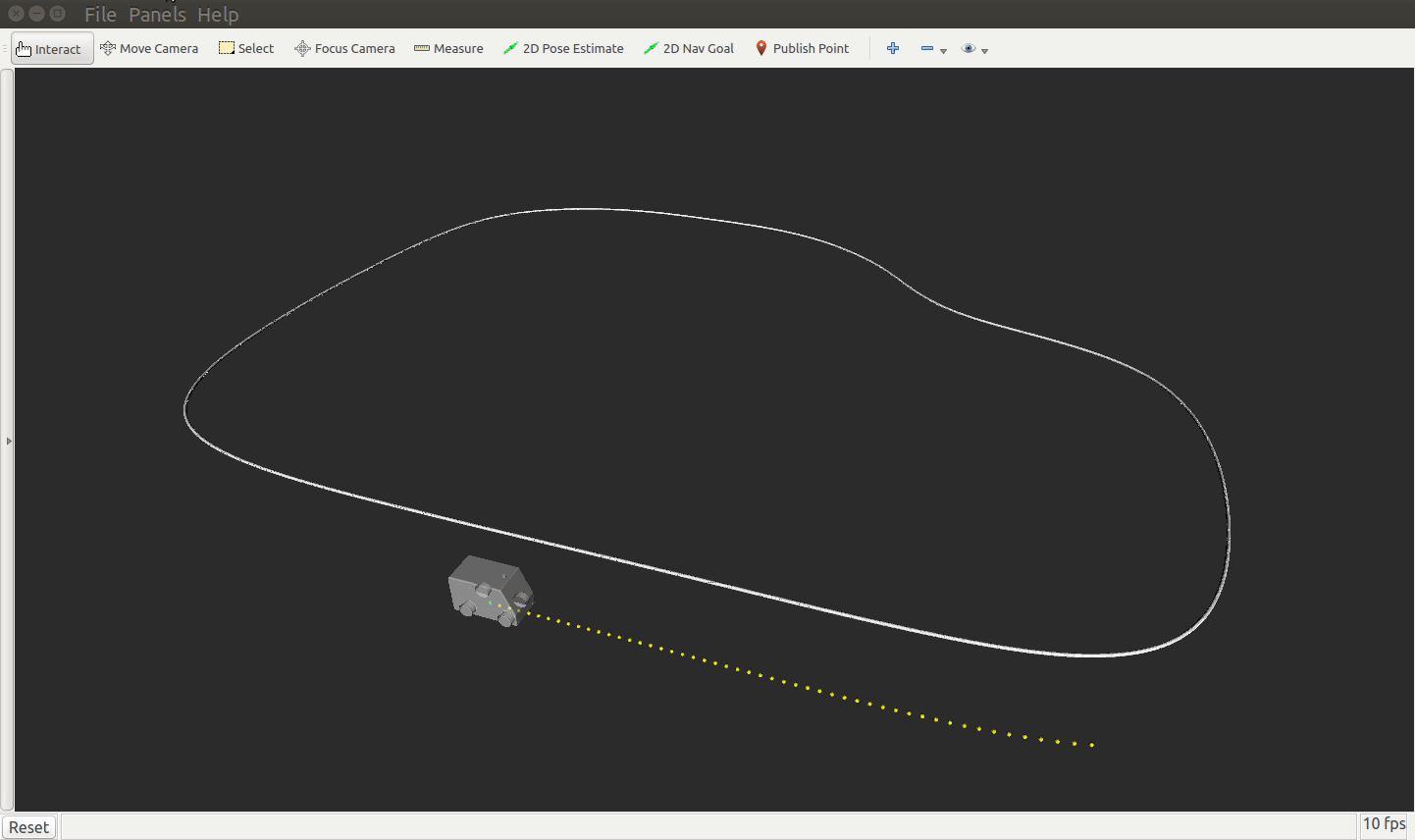1 前言
Frenet坐标系下的无人车轨迹规划方法（简称Frenet方法）是 Moritz Werling 在2010年的论文《Optimal Trajectory Generation for Dynamic Street Scenarios in a Frene´t Frame》中提出的，由于其简单有效的特点被广泛采用，甚至Matlab都设计了相应的函数：trajectoryOptimalFrenet，如下面左侧的动画。一些开源的无人驾驶项目也大量借鉴了方法中包含的思想，例如百度的Apollo无人驾驶项目，其中有很多Frenet坐标系的影子。 　　网上已经有很多Frenet方法的开源代码，我们后面的实现也基于公开的开源代码项目：SDC_ND_T3P1_Path_Planning，它既有Matlab的仿真代码也有C++的代码。其中，比较有名的是Udacity推出的课程附带的源代码，而且课程还提供了模拟器，能够对无人车进行虚拟仿真。关于项目如何运行起来，这篇文章说的很清楚了 　　关于模拟器介绍几点。模拟器叫Udacity Self-driving Car Simulator，是Udacity的纳米学位课程中搭建的教学软件，使用Unity 3D游戏开发软件制作，界面如下右图。其中的黑车是无人车，车前的绿色线条就是规划出来的轨迹。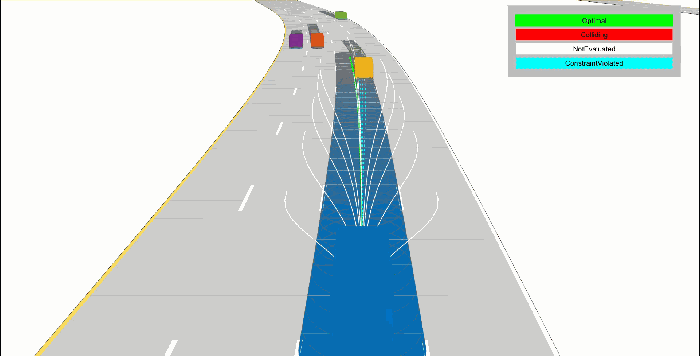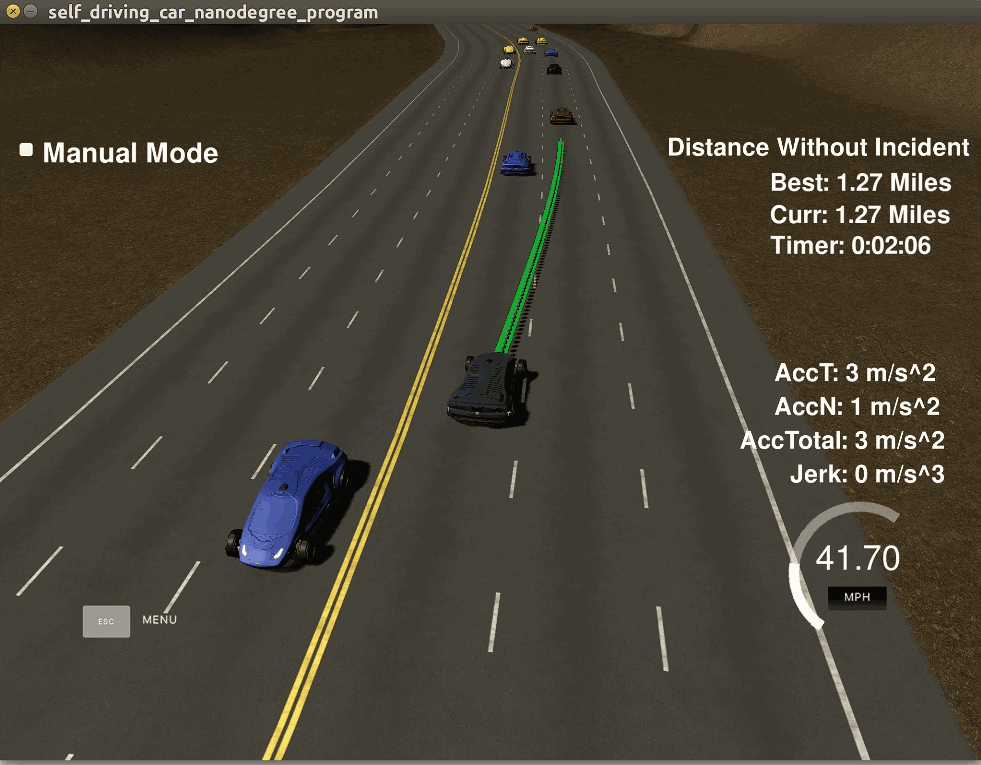模拟器是开源的，这篇文章提到，模拟器是用Unity 3D V5.5.1f1版本开发的，不要使用过高版本打开，因为老版本中的有些函数新版本并不支持。模拟器可以点击这里下载。但是笔者发现，有些资源（png材质）被禁止下载了，就算你安装了大文件版本git lfs，就算你fork到自己的仓库或者码云也不行，因为作者加了限制，只有企业版才有下载权限。这就导致无法再在Unity修改，此外模拟器运行起来很耗资源，而且每次启动之后其它车辆的位置都是随机的，无法复现一些bug，所以我放弃了使用模拟器而采用ROS的Rviz作为可视化界面。
2 Frenet方法讲解
2.1　规划目标
如果问给无人车规划轨迹的宗旨是什么？笔者认为一般有两个：安全和舒适。安全肯定是首要的，如果规划出来的轨迹导致撞车显然是任何人都无法接收的。至于舒适，则主要面向有人乘坐的无人车，如果无人车动不动就加速或者急刹车你坐在上面肯定会抱怨，当然对于拉货的车辆也不是说可以任意加减速，这对车辆的执行器件也是伤害。因此这两个指标是一般的规划方法都要考虑的。Frenet方法也考虑了这两个方面。
2.2　Frenet坐标系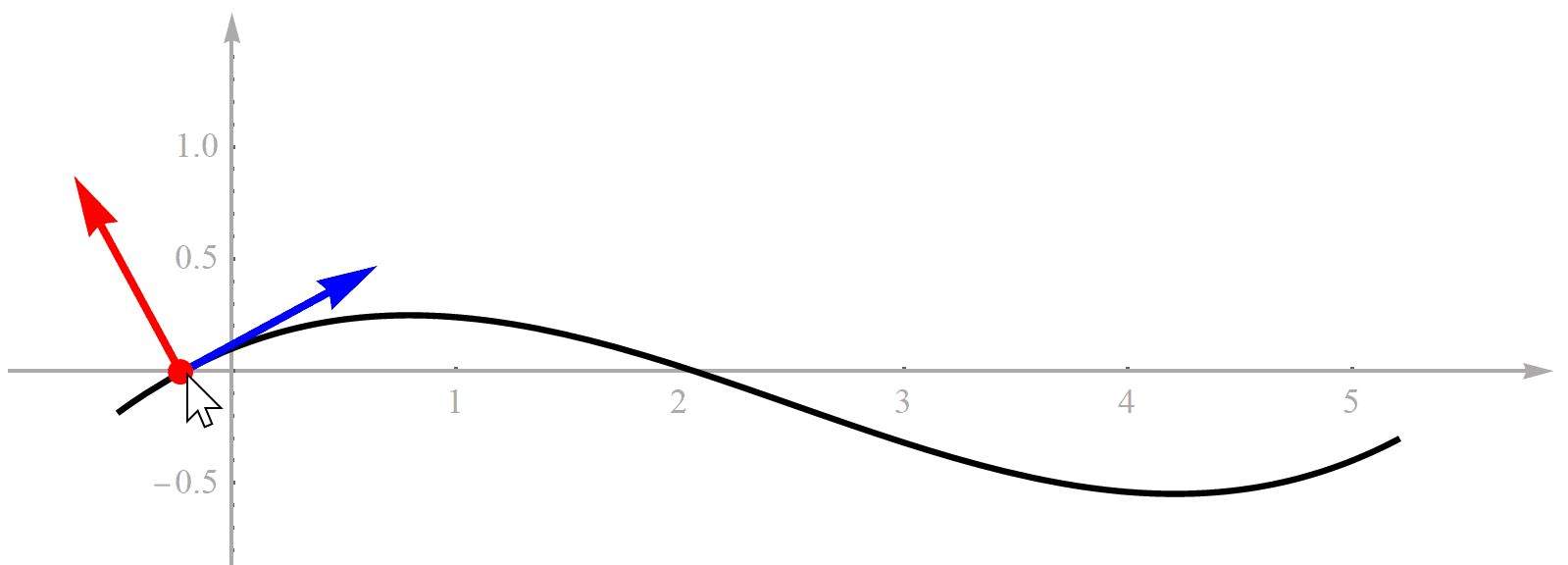你可能会问，既然Frenet坐标系的原点在道路参考线上，那么到底是哪个点？实际上，它是个动点。也就是说，它随着我们无人车的位置一直在变化。注意不要把Frenet坐标系理解成一个固定不动的全局坐标系，它是个移动坐标系。如果知道无人车在某个时刻的位置，那么曲线上有一个点到无人车的位置最近，这个点就是我们要的Frenet坐标系的原点。这里我们做一个假设，就是道路弯曲程度相对于无人车尺寸很小，所以只有一个点到无人车的位置最近，不会出现两个甚至更多距离同时都最小的点。 　　现在你知道了什么是Frenet坐标系？那么所谓的在Frenet坐标系下的轨迹规划又是什么意思呢？这其实就是说我们计算的多项式轨迹是相对于Frenet坐标系的，计算完后要再转化到一个全局直角坐标系中。用文字描述这个过程实在是不够直观，所以笔者又制作了一个动画，见下图。笔者用一个最简单的例子，就是一次多项式来展示。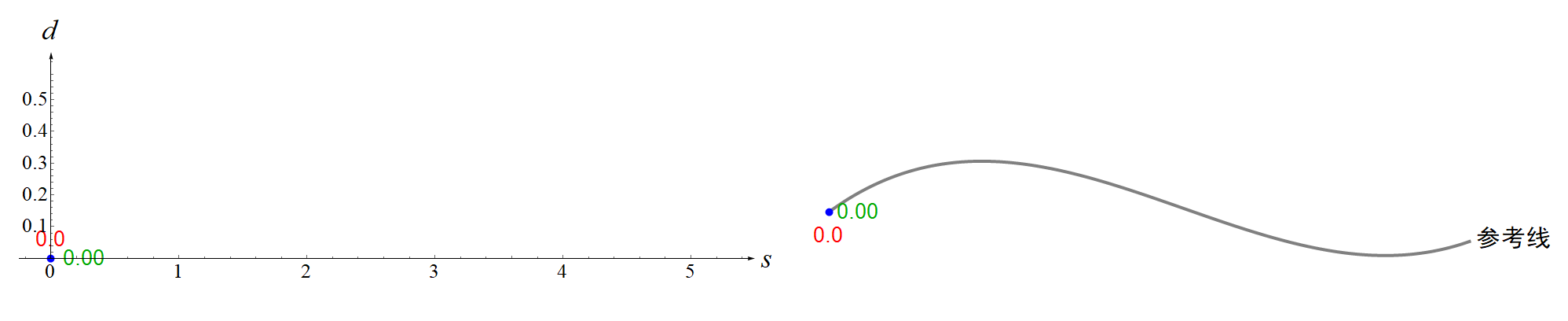特别注意，弧长

s

s

指的是道路参考线（center line）上的点与起点之间曲线的长度（也就是上图中的红色曲线），而不是你规划的轨迹（蓝色曲线）的长度。对于概念不清的初学者很容易弄混，笔者一开始也搞混了，导致看后面的推导公式总是理解不了。 　　所以，Frenet坐标系仅仅是一种描述方式，地位和全局直角坐标系一样。曲线的表达式与坐标系没关系，例如你可以在Frenet坐标系下使用任何一种曲线表达方式，例如B样条、贝塞尔曲线、Cycloid都可以，不必像原始论文一样局限于多项式。举个例子，华为的无人驾驶轨迹规划专利中就采用了分段样条曲线的表达方法：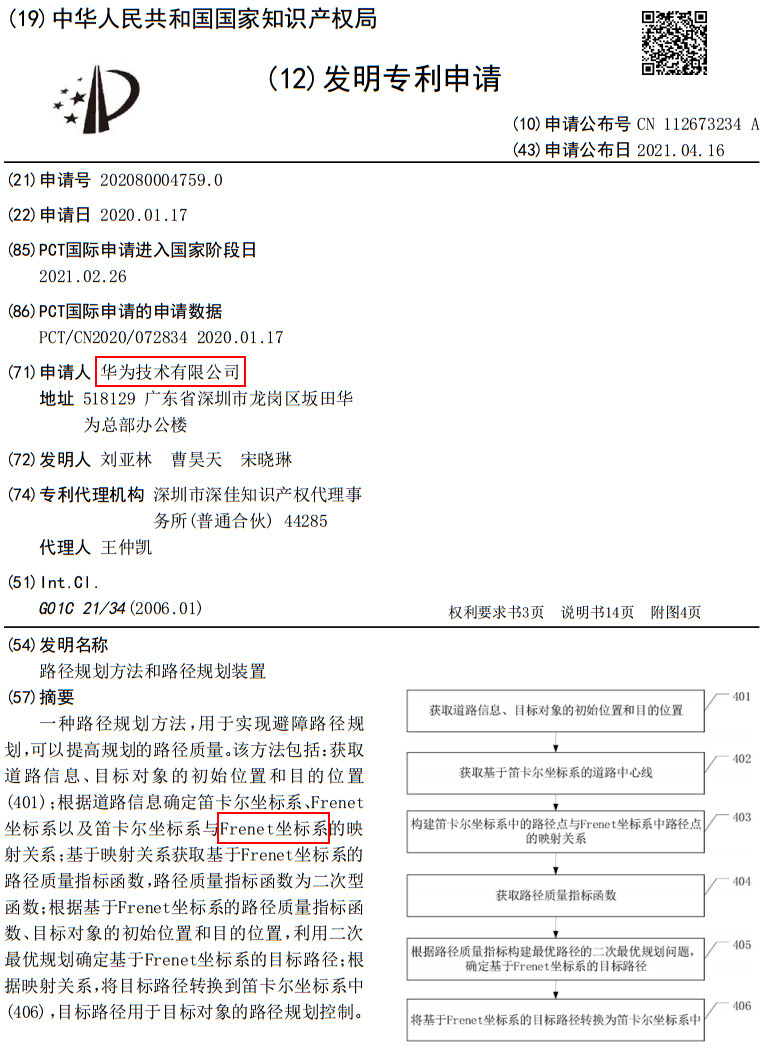2.3　参考线
Frenet坐标系是完全依赖参考线的，因此参考线在整个规划过程中是极其重要且不可或缺的。在百度Apollo中其被称为参考路径（Reference Line）。在轨迹规划之前要先生成参考线，那么参考线是怎么来的呢？一般是先根据起点和目标点进行路线规划，根据路线经过的道路从高精地图中查找对应的道路中心线或者边界线，将所有道路的道路中心线拼接起来并进行光滑处理，防止出现间断或者跳变。这样就得到了一条光滑的参考线。
2.4　多项式
这里说的多项式是指像这样

y

(

x

)

=

a

0

+

a

1

x

+

a

2

x

2

+

a

3

x

3

+

a

4

x

4

+

a

5

x

5

y(x)=a_{0}+a_{1}x+a_{2}x^{2}+a_{3}x^{3}+a_{4}x^{4}+a_{5}x^{5}

的一元幂次多项式。我们在初中就已经知道多项式了，对它的性质也比较熟悉。简单来说，无人车的轨迹规划就是生成一条曲线。我们可选的曲线并不是非常多，比如B样条曲线、贝赛尔曲线、还有我另一篇博客提到的Dubins曲线。如果画出函数的图像，多项式其实也是一种曲线。跟前面这些曲线相比，多项式的曲线其实更简单、直接。所以，为什么不试试用多项式来描述无人车的轨迹呢？显然论文作者就是这么考虑的。那么用几次多项式好呢？如果次数太少，曲线的表达能力不足，次数太多，曲线过于弯曲，也不容易求解。作者提出，使用4次和5次两种多项式。为什么选择这两种？作者给出了理由，首先，决定乘坐舒适与否的不是看速度、也不是看加速度、而是取决于加加速度（叫Jerk）。对曲线上的加加速度进行积分，值最小的曲线就是5次多项式。其次，5次多项式有6个待定系数，如果给定3个初始时刻的位置、速度、加速度三元组和终止时刻的位置、速度、加速度三元组这六个已知量，刚好可以求出6个待定系数从而完全确定这个多项式。当然，有时我们不关心速度，所以有时也用4次多项式。上篇博客提到，对于任意给定的两组位姿（初始位姿和目标位姿），它们之间总是有唯一的一条Dubins曲线。同样的，对于任意给定的两组位姿（也包括速度和加速度），它们之间也有一条唯一的5次多项式曲线。
2　Frenet坐标系与笛卡尔坐标系转换
轨迹规划就是计算多项式，这个过程是在Frenet坐标系中进行的。而计算轨迹的最大速度、判断有没有碰撞显然必须要在笛卡尔坐标系中进行，所以不可避免要在这两个坐标系之间转换。转换的方法如下： 　　1　Frenet

→

\rightarrow

Cartesian：即已知

(

s

,

d

)

(s,d)

坐标计算

(

x

,

y

)

(x,y)

坐标。这个计算简单，最开始用三次样条曲线（spline）对道路中心参考线参数化，具体就是用弧长

s

s

作为自变量，参考线的

(

x

r

,

y

r

)

(x_r,y_r)

坐标作为

s

s

的函数，即

x

r

=

x

r

(

s

)

,

y

r

=

y

r

(

s

)

x_r=x_r(s),y_r=y_r(s)

。根据

s

s

算出参考线的

(

x

r

,

y

r

)

(x_r,y_r)

坐标，再对样条曲线求导算出

(

x

r

,

y

r

)

(x_r,y_r)

处参考线的单位切线，再逆时针旋转

90

°

90 \degree

得到单位法线向量

n

n

，所以最终

(

x

,

y

)

=

(

x

r

,

y

r

)

+

d

n

⃗

(x,y)=(x_r,y_r)+d\vec{n}

； 　　2　Cartesian

→

\rightarrow

Frenet：即已知

(

x

,

y

)

(x,y)

坐标计算

(

s

,

d

)

(s,d)

坐标。这个问题不容易，相当于在曲线上找到一个点，使得它离曲线外面一个固定点的距离最小。当然，如果这个曲线非常复杂扭曲，找起来确实很难。但是曲线（参考线）是通过三次样条曲线插值离散参考点得到的，所以它就是个三次多项式，而且我们假设每两个参考点之间一段的曲线要么是直线要么是凸的，不会出现两次扭曲。当参考点取的足够密时，这样的假设是合理的。所以现在曲线的形式简单了，这样我们可以通过解方程求出这个点。 　　假设曲线外的点的坐标是

(

p

x

,

p

y

)

(p_x,p_y)

，三次曲线方程是

y

=

a

x

3

+

b

x

2

+

c

x

+

e

y=ax^3+bx^2+cx+e

，点到曲线的距离平方如下（记为

g

(

x

)

g(x)

）：

g

(

x

)

=

(

x

−

p

x

)

2

+

(

a

x

3

+

b

x

2

+

c

x

+

e

−

p

y

)

2

g(x)=(x-p_x)^2+(ax^3+bx^2+cx+e-p_y)^2

其中唯一的未知量是

x

x

，所以我们求

g

(

x

)

g(x)

的导数，并令其等于0即可。求出的点最多有五个，但是前面假设曲线只有一个弯曲的方向（三次项的系数

a

=

0

a=0

），所以实际最多三个点，我们挑出距离最小的那个即可。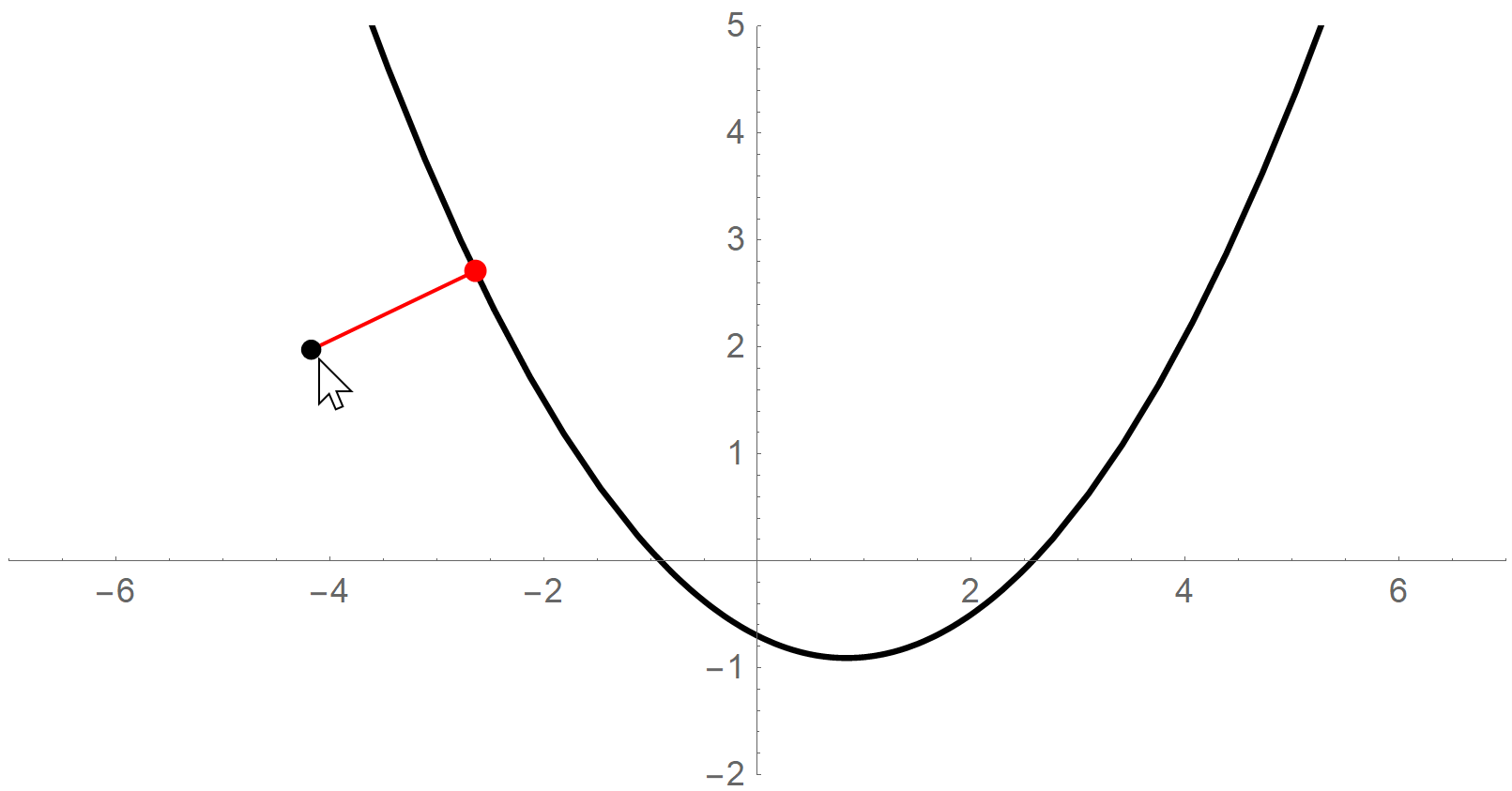注意，弧长

s

s

总是正的，但是

d

d

可以是负的，道路左侧可以定义成

d

>

0

d>0

，右侧可以定义成

d

<

0

d<0

。 　　在百度Apollo无人驾驶项目中，这二者的转换函数在modules\common\math路径下的cartesian_frenet_conversion.cc文件。但是cartesian_to_frenet函数只是把

d

d

算出来了，而最难的

s

s

没算（直接作为已知输入）。注意百度把

(

s

,

d

)

(s,d)

坐标记为

(

s

,

l

)

(s,l)

坐标，这是因为符号

d

d

被用于表示求导，防止弄混。
JMT函数中的polyeval(s0,T)是什么意思？polyeval是求多项式在某点的所有导数。这里的多项式是由系数s0定义的，s0是个状态类，它其实是

(

s

0

,

s

˙

0

,

1

2

s

¨

0

)

(s_0,\dot s_0,\frac{1}{2}\ddot s_0)

。所以对应的多项式就是按照系数从低到高：

f

(

t

)

=

s

0

+

s

˙

0

t

+

1

2

s

¨

0

t

2

f(t)=s_0+\dot s_0t+\frac{1}{2}\ddot s_0t^2

。这时再经过polyeval得到的输出就是三个导数项：零阶导数

s

0

+

s

˙

0

T

+

1

2

s

¨

0

T

2

s_0+\dot s_0T+\frac{1}{2}\ddot s_0T^2

、一阶导数

s

˙

0

+

s

¨

0

T

\dot s_0+\ddot s_0T

、二阶导数

s

¨

0

\ddot s_0

。 　　所以B就是两个三维状态向量之差：

s

1

−

(

s

0

+

s

˙

0

T

+

1

2

s

¨

0

T

2

)

s

˙

1

−

(

s

˙

0

+

s

¨

0

T

)

s

¨

1

−

s

¨

0

s_1-(s_0+\dot s_0T+\frac{1}{2}\ddot s_0T^2) \dot s_1-(\dot s_0+\ddot s_0T) \ddot s_1-\ddot s_0

generate_函数代码比较多，既然多我们就切成几部分来看。首先第一部分生成所有侧向轨迹，第二部分自然而然就是生成所有横向轨迹。第三部分则是将前面两部分的轨迹组合得到完整的轨迹，然后再根据代价从小到大排序，最后剔除掉可能碰撞的轨迹，最后剩下的最小轨迹就是输出的轨迹。
两个向量的叉积可以用来判断一个向量在另一个向量的那一侧，叉积的赋值是夹角的正弦。
{v1,v2}=m=RandomReal[{0,1},{2,2}];
ArcSin[Det[m]/Norm[v1]/Norm[v2]]
VectorAngle[v1,v2]
Graphics[{Arrow[{{0,0},v1}],Red,Arrow[{{0,0},v2}]},PlotRange->2]

3　行为决策
在行为决策上，作者将所有可能总结为4类：纵向方向的模式分别是Following，Merging，Stopping和Velocity Keeping。注意这里只提到纵向方向（也就是s坐标维度）。至于变道还是不变道则由侧向方向决定。侧向方向可以通过采样不同的侧向位置实现。此外，受到五次多项式本身表示能力的限制，规划出来的轨迹只能实现有限的行为，例如可以实现变道，但是无法生成连续的变道再回到原来车道的一段轨迹。 　　根据末端状态，前三种Following，Merging，Stopping可以归为一类，Velocity Keeping单独归为一类。为什么呢，因为前三种都需要限制轨迹末端的位置、速度和加速度，而Velocity Keeping只需要限制末端的速度和加速度，对位置不限制。从多项式函数的角度看，前三种用五次多项式表示，后一种用的是四次多项式。所以在代码中定义了following_merging_stoping函数统一处理。对于侧向方向不作分类，总是五次多项式，因为我们一定要约束侧向方向的末端位置在车道某处，而不希望无人车自由发挥。在实际应用中，笔者认为Merging应该很少遇到，所以可以不考虑，本文也不过多讨论。对于Following和Stopping来说，我们都要明确知道前车的状态（主要是位置和速度）。作者只考虑了Following，Merging和Velocity Keeping。Merging出现最少，只出现了2次。Following和Velocity Keeping分别都出现了5次。 　　下面重点分析Following和Stopping。 　　Stopping：在原程序中，作者没有考虑Stopping这种情况（所以TrajectoryGenerator.h中的stopping函数始终没被调用过）。当然这也是有道理的，因为无人车在正常执行任务时，我们希望Stopping最好不要出现，除非遇到特殊情况不得不停车，这时无人车可能无路可走了。 　　Following：跟车时，我们最关心的决策信息是被跟随者的位置和速度。首先，被跟随者必须在无人车的前方，如果被跟随者与无人车齐头并进或者在无人车后面，那显然无人车不能跟随它。如果只考虑速度还不够，如果被跟随者的速度为0甚至为负，那么无人车显然应该避让，不能再跟随。所以跟随模式的条件可以设计成下表这样的形式。其中，

s

c

s_c

是被跟者的纵向位置，

s

e

s_e

是无人车的纵向位置，

v

c

v_c

是被跟者的纵向速度。只有当间距足够大和速度足够快同时满足，无人车才会考虑跟车。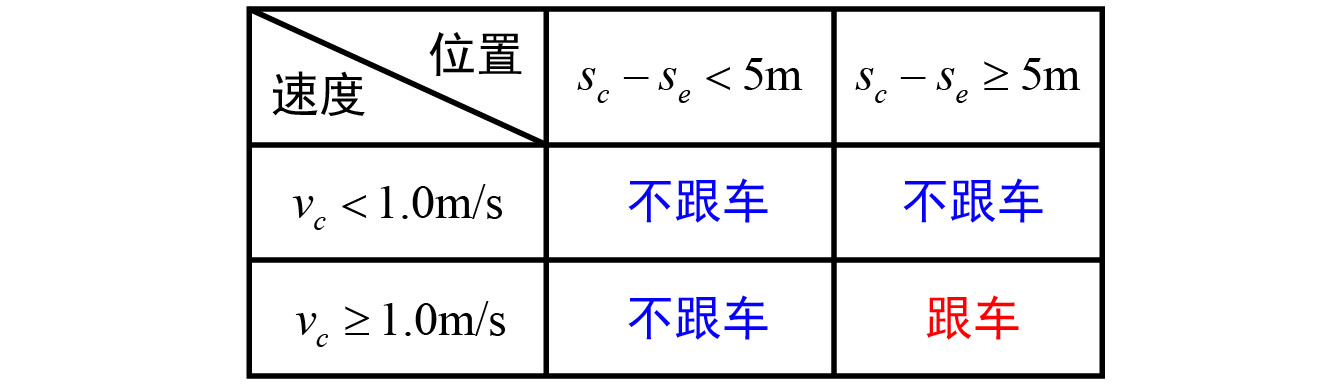BehaviorModule.h文件，其中Search_Center函数用于搜索无人车自己所在车道的情况，并以此进行决策，决定是采用FOLLOWING还是VELOCITY_KEEPING亦或MERGING。决策过程的判断逻辑是如下： 　　首先，查看目标车道Goal_Lane的前后有没有其它车辆，分成两种情况： 　　1 前面有车，此时根据后面有没有车再分成两种情况： 　　　　1.1 后面有车，此时计算前后车的间距，称为自由空间free_space。如果自由空间比Max_Tight_Merge_Offset大，再看与汽车的距离nf，如果nf小于Consider_Distance，则采用FOLLOWING模式， 采用前车的速度跟车行驶。如果nf不小于Consider_Distance，则采用VELOCITY_KEEPING模式，以v_ref参考速度速度保持。如果自由空间不比Max_Tight_Merge_Offset大，而是只比Min_Tight_Merge_Offset大，说明自由空间不宽裕，此时采用MERGING模式，保持在前后车中点位置行驶。如果自由空间比Min_Tight_Merge_Offset还小，此时不管，该情况应该是危险的特殊情况，应该单独考虑。 　　　　1.2 后面没车，此时如果无人车与前车的距离小于Consider_Distance，则采用FOLLOWING模式， 采用前车的速度跟车行驶。如果与前车的距离大于Consider_Distance，则采用VELOCITY_KEEPING模式，以恒定的v_ref参考速度。 　　前面有车时还做了一件事，就是看前车的车速，如果车速小于v_ref，说明前车太墨迹开得太慢，那么就要考虑变道超车，将变道超车的标志位attempt_switch置为true。 　　2 前面没车，以v_ref参考速度速度保持。此时不管后面有没有车，后面有车也不理它。 　　乍一看，上面的模式好像很多，有些复杂，但是实际上这些条件判断都是排他性的，所以最后只能有一种模式被选中，不会同时选择多个。最后把搜索模式和attempt_switch返回。
如果后方来车比较快，无人车应该怎么反应？
最核心的PlanPath函数主要起到行为决策的功能，它只有两个输入，无人车自身的实时信息ego（包括尺寸、状态、轨迹）和无人车附近的其它车辆near_cars的信息。注意它除此以外没有接收其它信息，所以这个函数只能无休止的跑下去，而不能达到某个期望的目标位置，这显然是需要改造的。我们先分析PlanPath函数的功能： 　　1 首先，根据无人车的横向距离计算所在车道ego_lane，再计算无人车的纵向速度ego_speed。再有就是设定无人车的参考车速v_ref，这是非常重要的部分，笔者认为这部分不应该藏在这里，而是应该作为输入参数，因为我们可能会经常根据环境和任务调整这个参考速度。 　　2 随后，从其它车辆near_cars中提取其它的信息，例如每辆车所在的车道、和无人车的间距、预测的纵向车速等等。 　　3 最后也是最重要的部分，就是利用前面提取的信息搜索行为模式了。为了表示模式，作者定义了SearchMode和ActiveMode结构体。搜索出行为模式后，后面的函数会从这两个结构体中提取出行为类型和与这种行为有关的参数，例如目标速度、前车的状态等等，进行具体的轨迹生成。所以为了理解后面的程序，就要先了解这两个结构体的定义。 　　ActiveMode结构体中定义了三个成员，如下： 　　①　具体的行为模式（Following，Merging，Stopping，Velocity Keeping其中的一种） 　　②　其它车辆的状态，包括无人车前方和后方的车辆 　　③　数字。最后这个数字number有两个模式会使用到，分别是停车和速度保持，当然对应的含义也不一样。对于停车模式，number存储的是应该停车的目标位置（纵向位置s）；对于速度保持，number存储的当然就是目标车速了（纵向速度）。这些在TrajectoryGenerator.h文件中的generate_函数中可以根据函数调用很容易猜出来。
struct ActiveMode {
Mode mode;
vector<State> state;
double number;   };

SearchMode结构体中也定义了三个成员，分别是： 　　①　ActiveMode结构体　　你可能注意到了，activeModes不是一个，而是一个向量，也就是说SearchMode结构体可以存储不只一个ActiveMode结构体。那么对于多个SearchMode结构体，它们中的具体模式有没有可能重复呢，不排除这种可能。 　　②　目标车道　　　　　　每个SearchMode只存储一个目标车道。 　　③　最小前车速度　　　　min_lv_speed只在BehaviorModule.h文件中使用，其它文件中没有见到使用。min_lv_speed用来计算轨迹的代价。
struct SearchMode {
vector<ActiveMode> activeModes;
double goal_lane;
double min_lv_speed;   };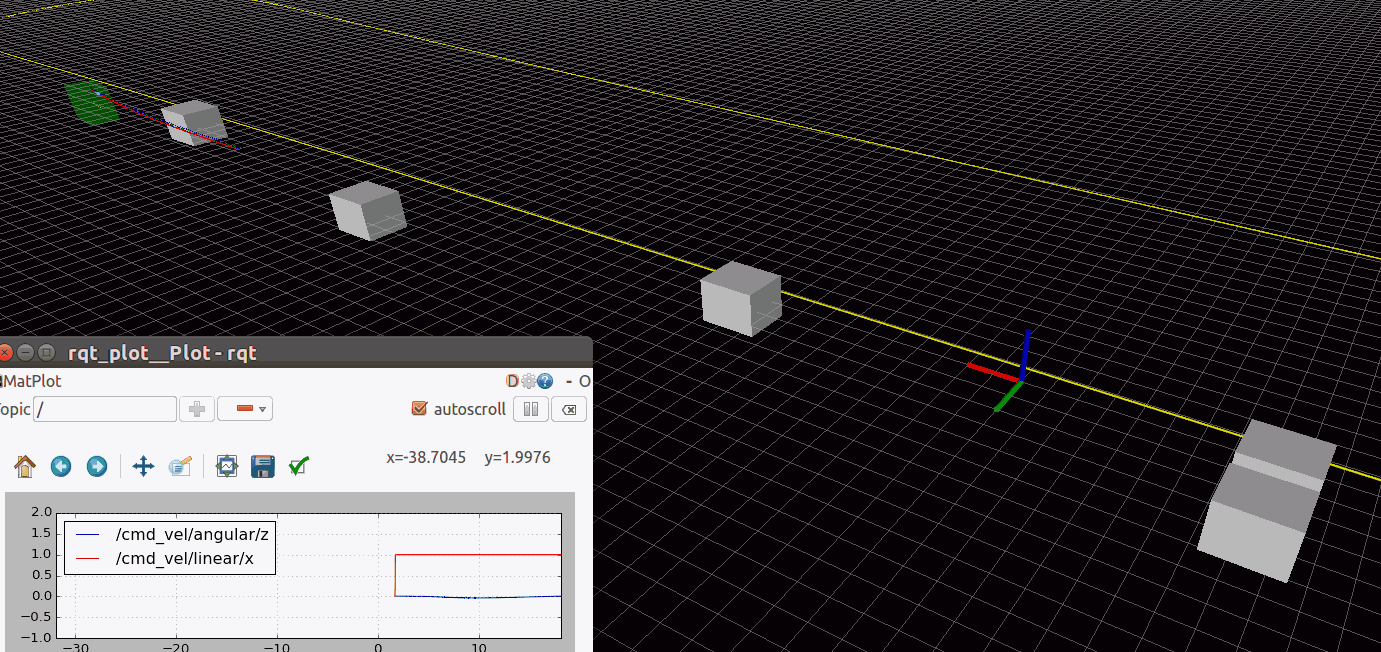3　多项式函数库polynomial.h
文件polynomial.h包含一系列与多项式有关的函数，例如相乘、求导等，其命名与MATLAB一样。polyval函数的作用是计算某个（x）值处的多项式函数值（y(x)），其定义如下。MATLAB也有同名函数。
/* polyval : evaluate a polynomial at xi */
double polyval(VectorXd const& coef, double xi) {
int N = coef.size();
if (N==1) return coef(0);
double yi = coef(N-1);
for (int i=N-2; i>=0; --i) {
yi = (yi*xi + coef(i));
}
return yi;
};

polyval函数的输入是定义多项式的系数coef和自变量取值x。系数coef的定义是低次项在前，高次项在后。我们举个例子，假设系数coef = {a, b, c, d};，那么x处的函数值是y(x) = a + b x + c x^2 + d x^3。在Mathematica软件中可以看到计算结果：
coef={a,b,c,d};
n=Length[coef];
y=coef[[n]];
For[i=n-1,i>=1,--i,y=(y*x+coef[[i]])]
y//Expand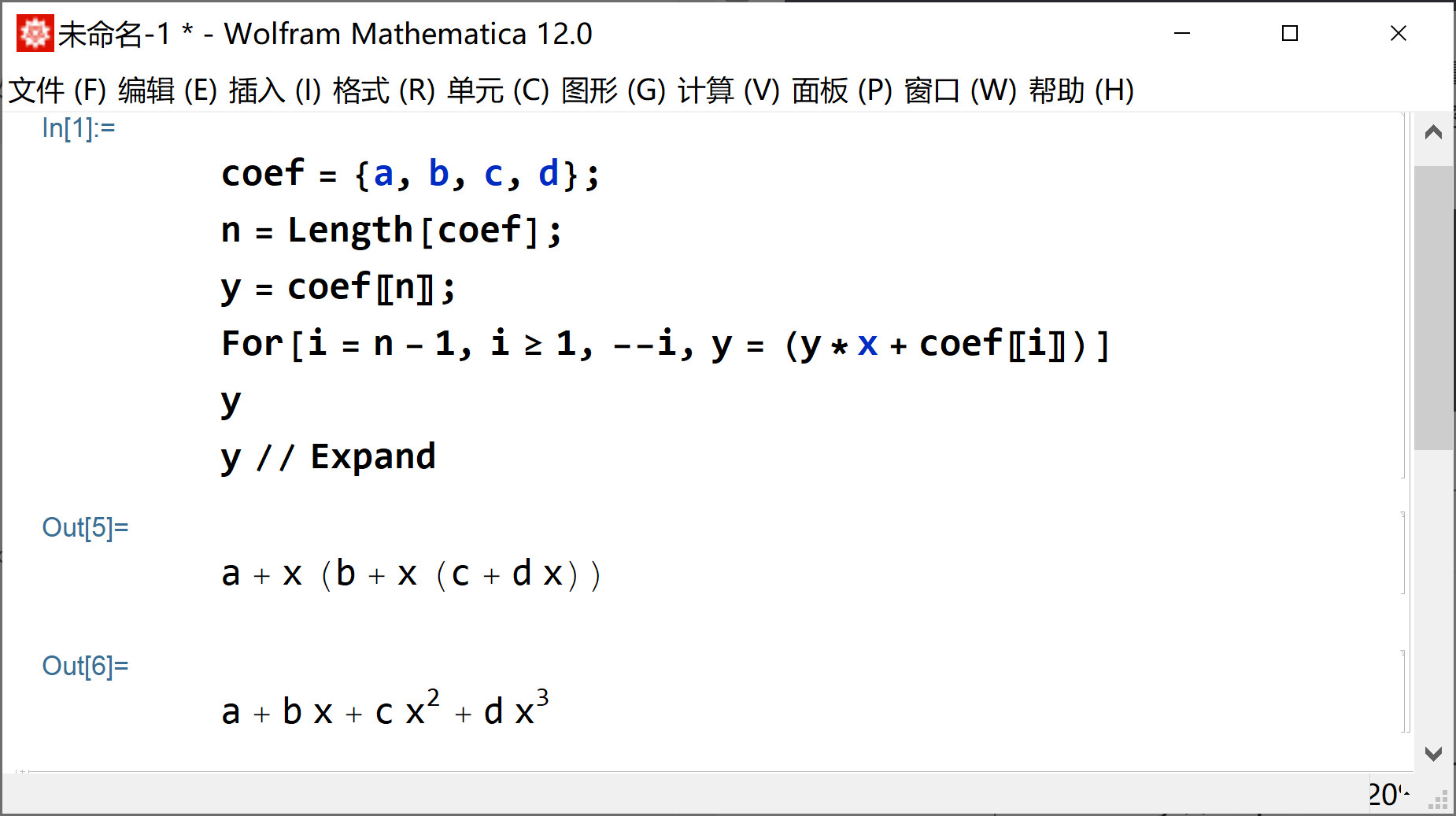另一个长得差不多的函数polyeval的功能是计算多项式在某点处所有的的导数。注意是所有的导数，包括0阶导数（就相当于计算函数值），以及无穷阶导数（超过次数+1以后都是零）。
VectorXd polyeval(VectorXd const& coef, double xi) {
int N = coef.size();
// Compute a factorial array and the powers of xi
VectorXd fac = VectorXd::LinSpaced(N,0,N-1);
VectorXd xip = VectorXd::Ones(N);
fac(0) = 1;
if (N>1) {
xip(1) = xi;
for (int i=2; i<N; ++i) {
fac(i) *= fac(i-1);
xip(i) = xip(i-1)*xi;
}
}

xip = xip.cwiseQuotient(fac); // xi^j/j!
VectorXd f = coef.cwiseProduct(fac); // a(j) * j!

// The n'th derivative at xi is a convolution between f and xip
for (int r=0; r<N; ++r) {
}
};

PP的意思是分段多项式函数（Piece-wise polynomial），作者单独定义了一个类来表示该函数。具体来说，PP就是一个多次样条曲线，而PP2是PP的一个特例，是2段多次样条曲线。它们都在polynomial.h里，Trajectory类就是继承自PP2，说明它也是一个“2段多次样条曲线”，但是Vehicle中出现的Trajectory最多只使用二次样条，表示位置、速度和加速度，这是初始化时决定的。所以如果运行Vehicle中的display()会看到trajectory.display();只显示三个数值（代表多项式系数）。正常的四次或五次多项式则会显示5个或6个数值。
Trajectory() : PP2() {};
PP2() : coef({VectorXd::Zero(3)}), knot(1e300) {}

VectorXd::Zero(3) = {0,0,0}

4　Trajectory类
Trajectory类对两段分段多项式PP2类重新封装了一下，说白了就是用时间参数化的多项式来表示轨迹，这个轨迹可以是无人车本身的也可以是动态障碍物的。既然用时间参数化了，那就可以取出某个时刻物体（例如无人车）的状态，这里的状态具体指的是：位置、速度、加速度，程序中用Vector3d类型表示。注意一个轨迹Trajectory只是针对一个维度，而我们的物体用一个维度是不能确定的，必须用两个维度（就是对应Frenet坐标系的s维和d维）。所以在Vehicle类中定义了二维轨迹向量：vector<Trajectory> trajectory;
5　车辆类型
该程序定义车辆为Vehicle类，不管是无人车还是其它障碍物车辆都用一个类表示。如果再扩展一些，这个类也是对静态和动态物体的统一表达，因为静态可以被视为动态的特例，当然行人、自行车也可以使用这个类来表示。Vehicle中存储着车辆的以下信息： 　　①　ID标示 　　①　形状信息，将车辆用长方形表示，需要定义长和宽 　　①　状态信息，即位置、速度、加速度 　　①　轨迹信息，这个表示未来一定时间车辆可能的状态。 　　正因为有轨迹信息，所以支持对动态物体进行避障。碰撞检测函数是helpers文件中的overlap函数。compute_2d_validity函数遍历不同时刻无人车和障碍物的位置并调用overlap函数进行碰撞检测。
5　参考线
该项目必须先要有一个参考线，参考线的制作可以阅读spline.h文件，方法是对一些离散的点进行分段三次样条插值，得到一段段连续的解析曲线。RoadMap.h文件用于定义与参考线有关的计算函数，例如从frenet坐标转换为直角坐标，这个函数就是getXY。 　　定义参考线所需的离散点被弧长参数化，其格式如下。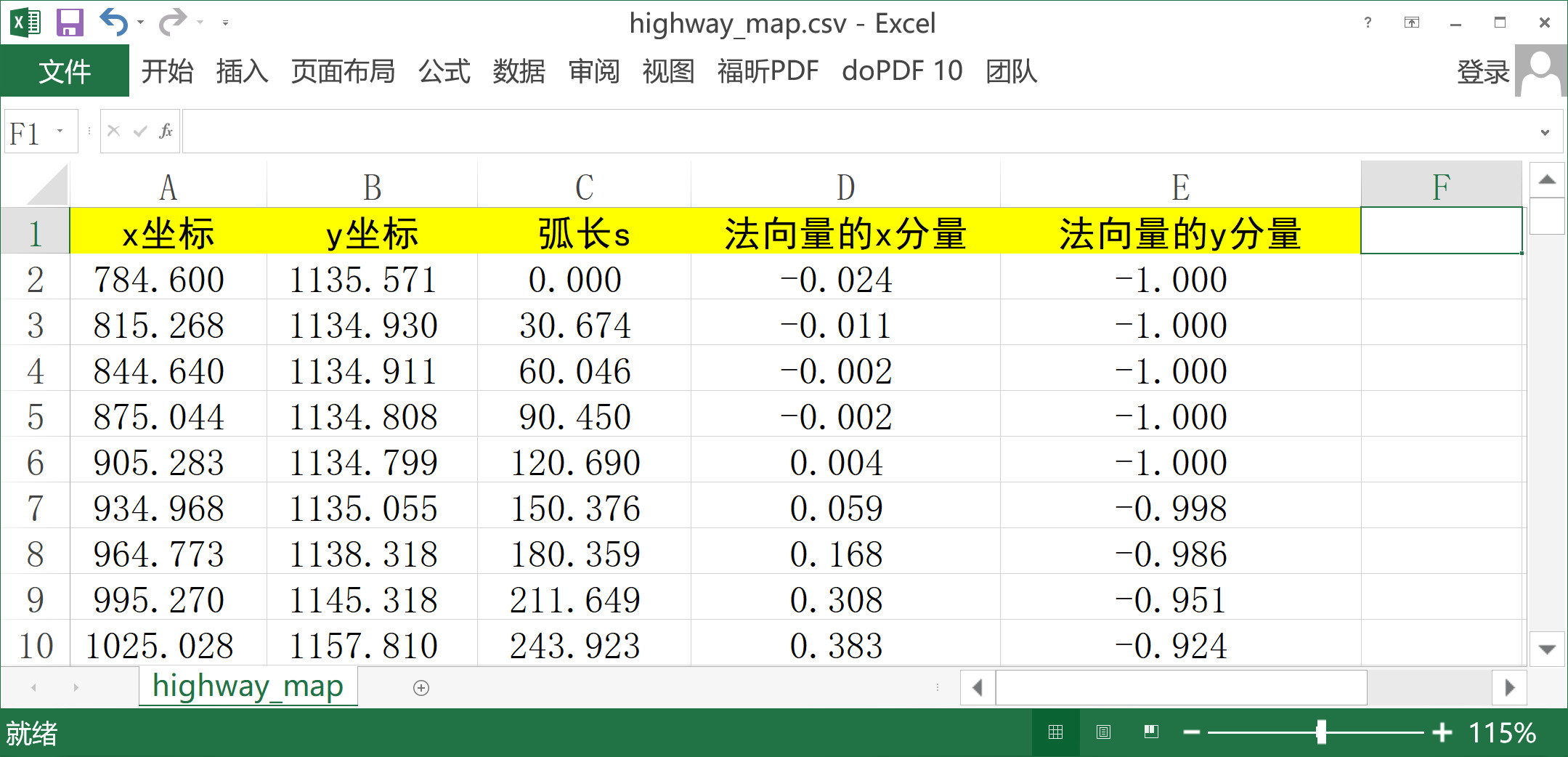如果在数学计算软件中显示出来就是下图这样的，红色的箭头就是法向量，每个点处都有一个。参考线应该怎么生成这个问题没有唯一答案。参考线可以位于道路中心，也可以位于道路最左侧或者最右侧，随你的习惯而定。不过原程序中默认是位于最左侧的，即无人车总是在参考线右侧行驶。如果想把参考线定义成成最右侧，可以改变getXY函数里的法向量的方向，加个负号即可，其它地方不用改。
    // road normal vector
Vector2d n;
n << t(1), -t(0);
r += -d*n; // 原来是r += d*n;

3 起点问题
轨迹规划的起点是什么？大多数人想当然的第一反应是：当然是无人车实际的位置了。但这会产生一个严重的问题，如下图所示。红色曲线就是规划的轨迹，这条轨迹在拐弯的时候将无人车导向了车道边缘，这显然不是我们想要的。为什么会出现这个问题呢？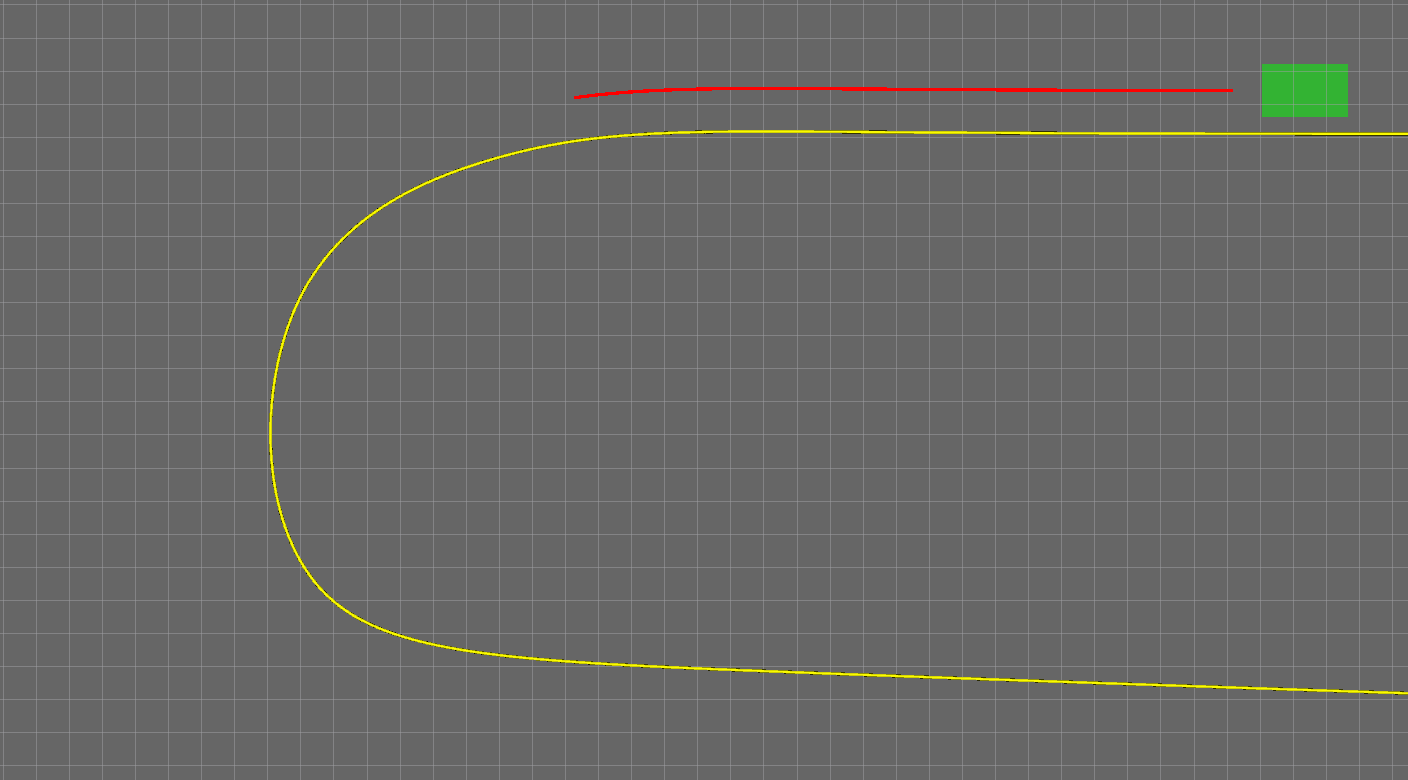所以，在设置规划的起点时一定要小心。
4 Rviz显示
4.1 C++编程
利用Rviz发送障碍物，演示避障。演示停车。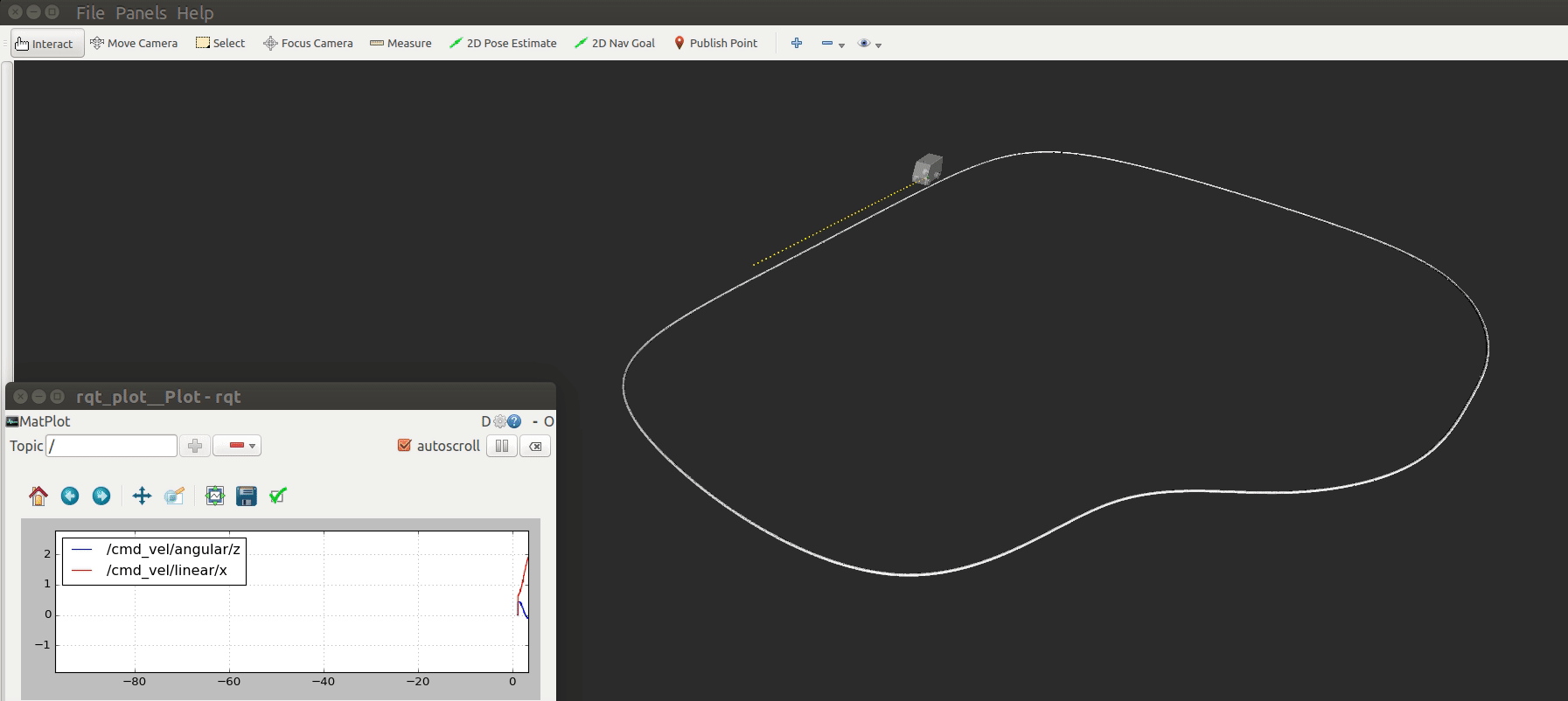5 踩过的坑
5.1 C++编程
5.1.1 在一个构造函数里调用另一个构造函数
第一个大坑是关于构造函数调用的，这个问题有人遇到过：C++中可以在构造函数中调用另一个构造函数吗？ 　　下面这样直接调用是不可以的，编译不会报错，但是一旦运行就有问题。
Vehicle::Vehicle(int id)
{
Vehicle(id, length, width);
}

但是写成这样是可以的，运行没有问题。
Vehicle::Vehicle(int id) : Vehicle(id, length, width, 0) {};

5.1.2 函数的默认输入
如果函数有默认的输入，声明和实现都要写。 　　头文件中写声明，注意C = 0：
VectorXd polyint(VectorXd const& coef, double C = 0);

cpp文件写实现：
VectorXd polyint(VectorXd const& coef, double C = 0) {
int N = coef.size();
VectorXd coefi = VectorXd::Zero(N+1);
coefi.tail(N) = coef.cwiseQuotient(VectorXd::LinSpaced(N,1,N));
coefi(0) = C;
return coefi;};

5.1.3 const
如果不想定义成类，那么成员变量怎么定义？在头文件中的变量定义时加上const修饰符即可，这样就能在cpp文件中使用了。
5.1.4 头文件中定义函数
头文件中不能定义函数，除非定义成内联函数或者加上const修饰符。
展开全文自动驾驶
• ## Frenet坐标系相关知识系统学习

万次阅读 多人点赞 2019-10-31 10:20:49
一、Frenet坐标系简介 二、Frenet坐标系与全局坐标系的转换 可以基于Frenet坐标系，报据自动驾驶车辆的始末状态，利用五次多项式建立自动驾驶车辆轨迹规划模型，并建立各个场景下的轨迹质量评估函数。 三、...

零、Frenet坐标系简介
自动驾驶汽车路径规划技术的难点之一在于规划过程中难以表达车辆与道路之间的相对位置,导致二者之间的相对关系不明确。因此,传统规划算法在笛卡尔坐标系下规划出的路径对于开放道路有良好的效果，但是对于公路环境，忽略车道信息导致路径的自由度太高而容易违反道路交通规则。在DAPRA汽车挑战赛期间，由斯坦福大学提出的路径规划算法将横向偏移(lateral oﬀset)定义为相对于基础路径(baseframe)的垂直距离。由于基础路径为道路中心线,这样的定义方式使得道路与车辆之间的关系更为直观。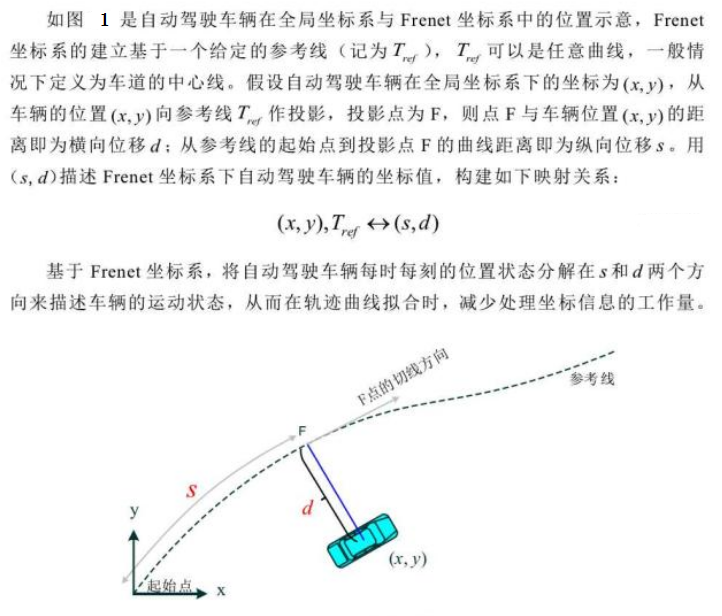一、Frenet坐标系与全局坐标系的转换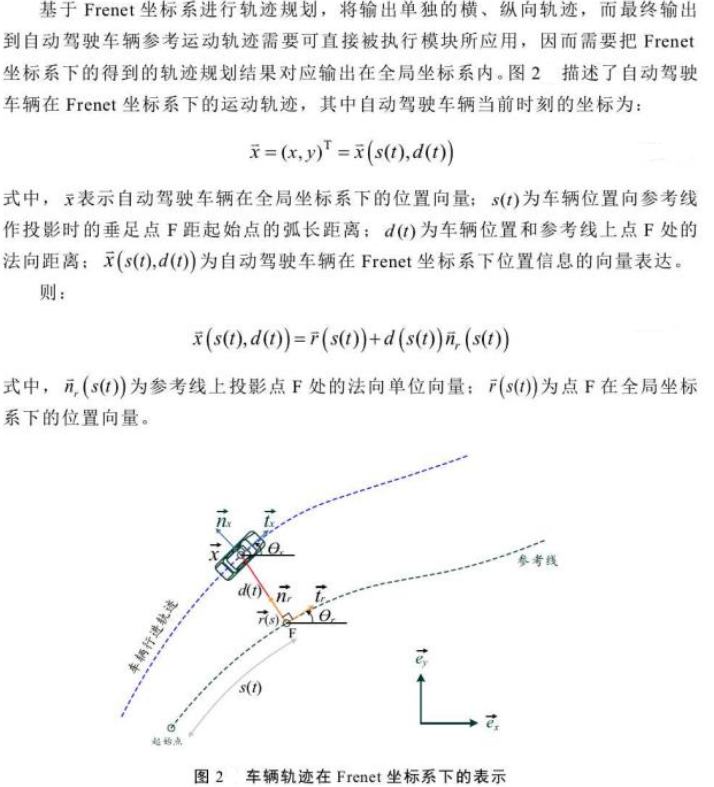Frenet坐标转化公式推导（Frenet 坐标系与全局笛卡尔坐标系转换）参考博客《轨迹规划1：Frenet坐标转化公式推导》
总结如下：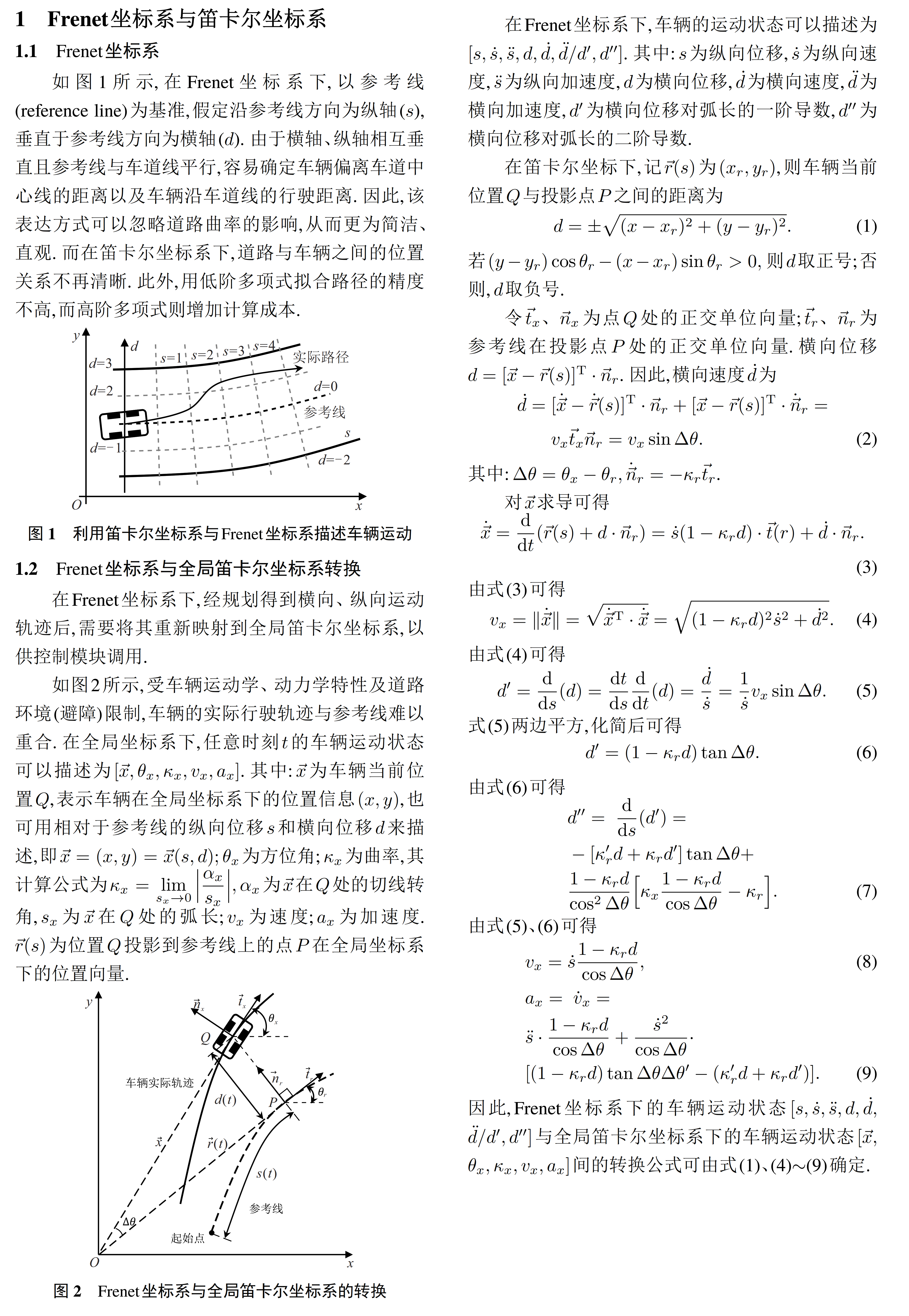（1）《无人驾驶汽车系统入门（二十一）——基于Frenet优化轨迹的无人车动作规划方法》
（2）《控制及决策》期刊2021论文《基于Frenet坐标系的自动驾驶轨迹规划与优化算法》

二、基于jerk 的实时（横纵向）轨迹规划求解
可以基于Frenet坐标系，报据自动驾驶车辆的始末状态，利用五次多项式建立自动驾驶车辆轨迹规划模型，并建立各个场景下的轨迹质量评估函数。 基于Frenet坐标系，提出一种能够面向公路场景的轨迹规划算法。首先，利用Frenet坐标系，将二维的车辆运动解耦成相互独立的一维纵向、横向运动，构建以 jerk 为核心的一维独立积分系统，利用5次、4次多项式分别求解得到有限的横向、纵向集合；然后，基于高斯卷积、加速度变化率，从安全性和舒适性设计损失函数以最小化轨迹成本；最后，通过模拟类公路场景验证算法性能，并开展轨迹优化选择以及采样优化等内容的研究。研究结果表明，该算法为车辆规划出的轨迹平滑、舒适、安全性更高。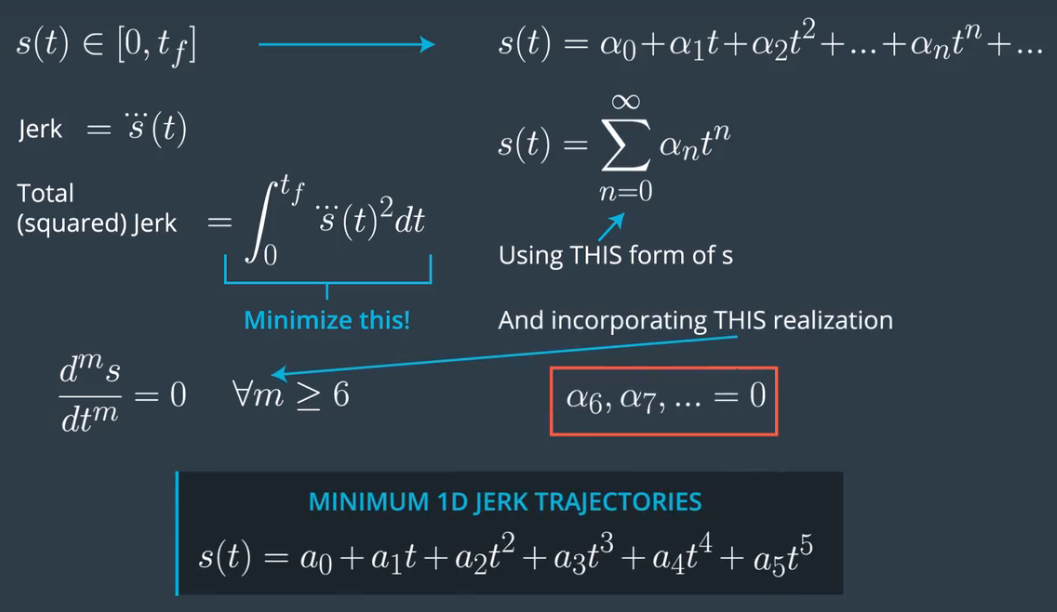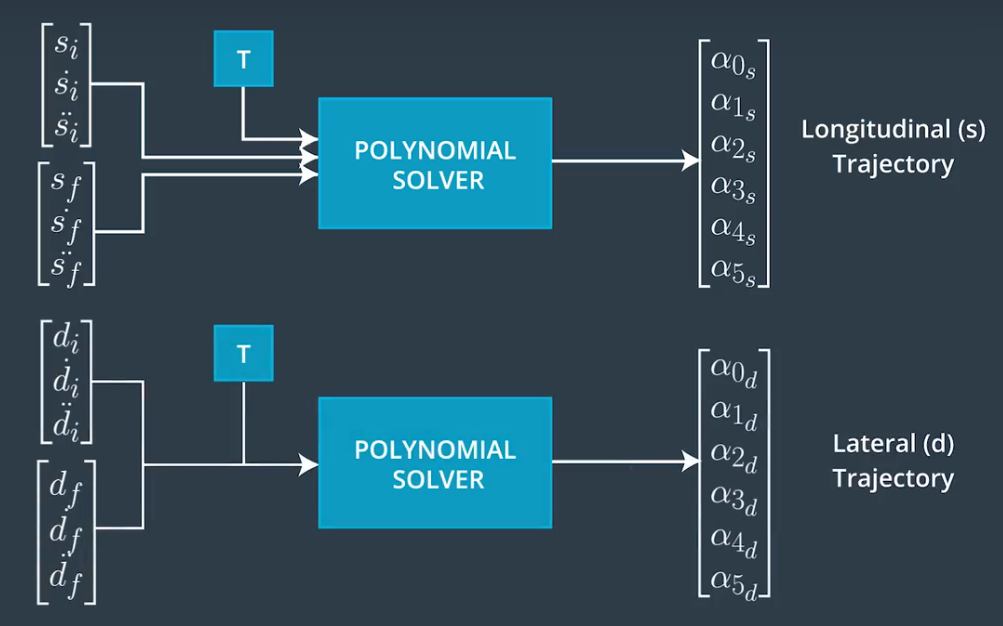2.1 基于jerk 的一维无约束积分系统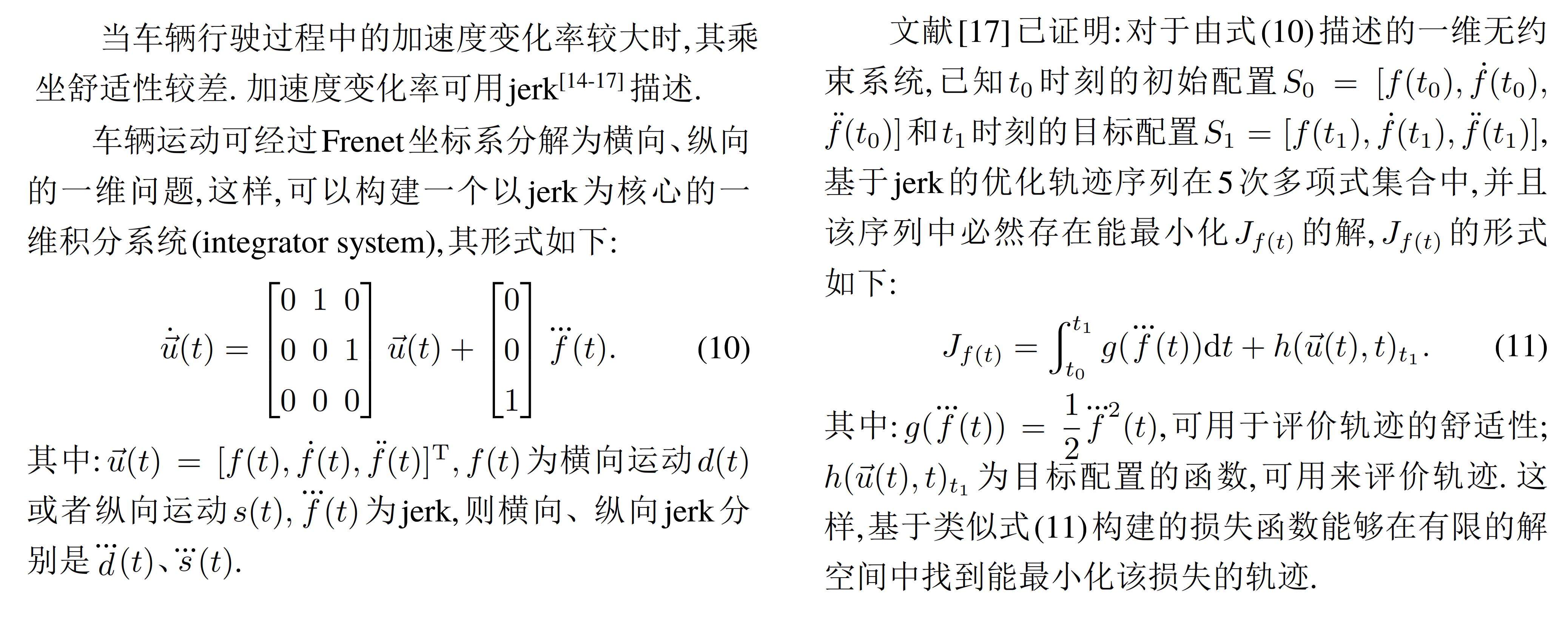2.2 横向轨迹规划求解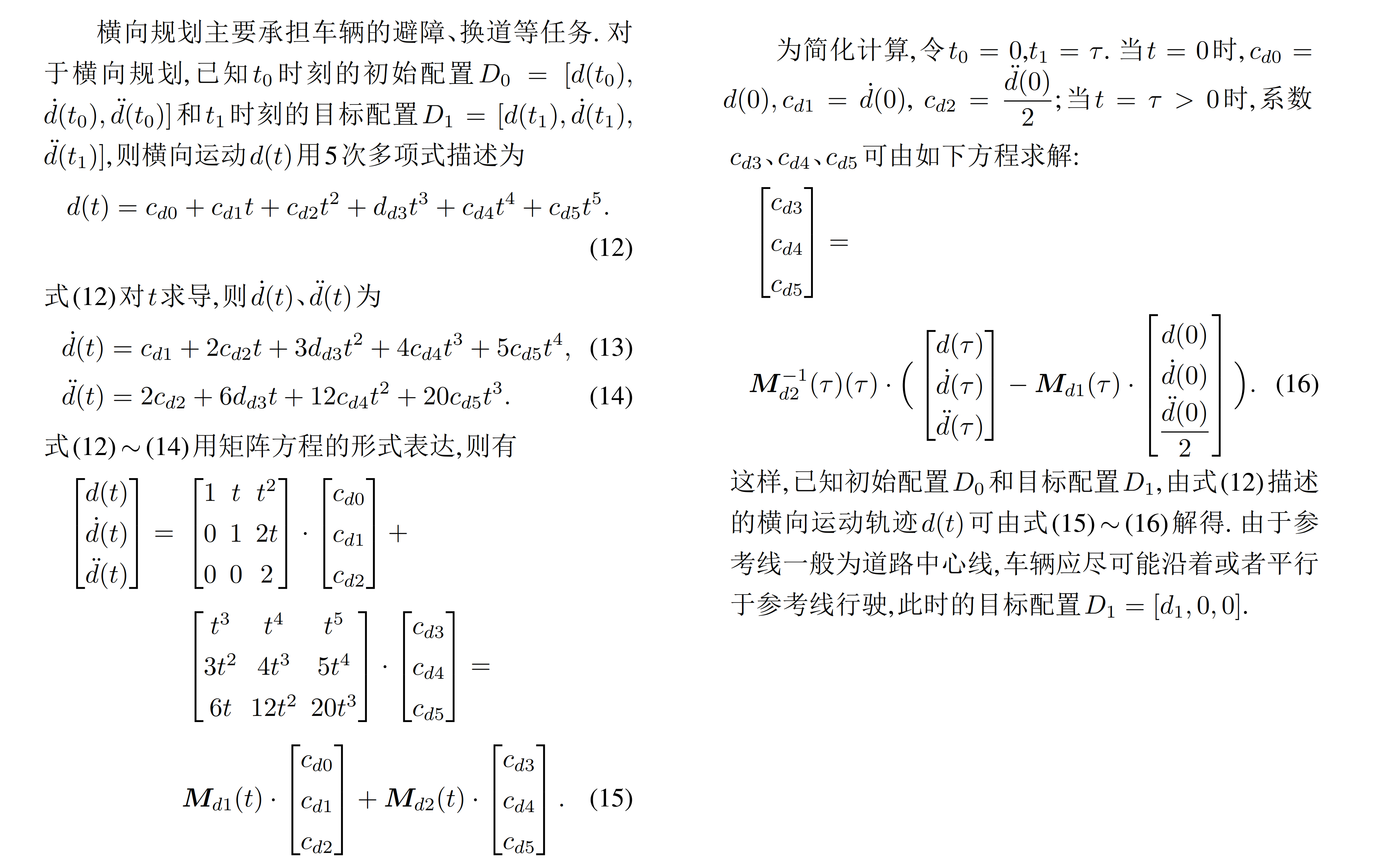2.3 纵向轨迹规划求解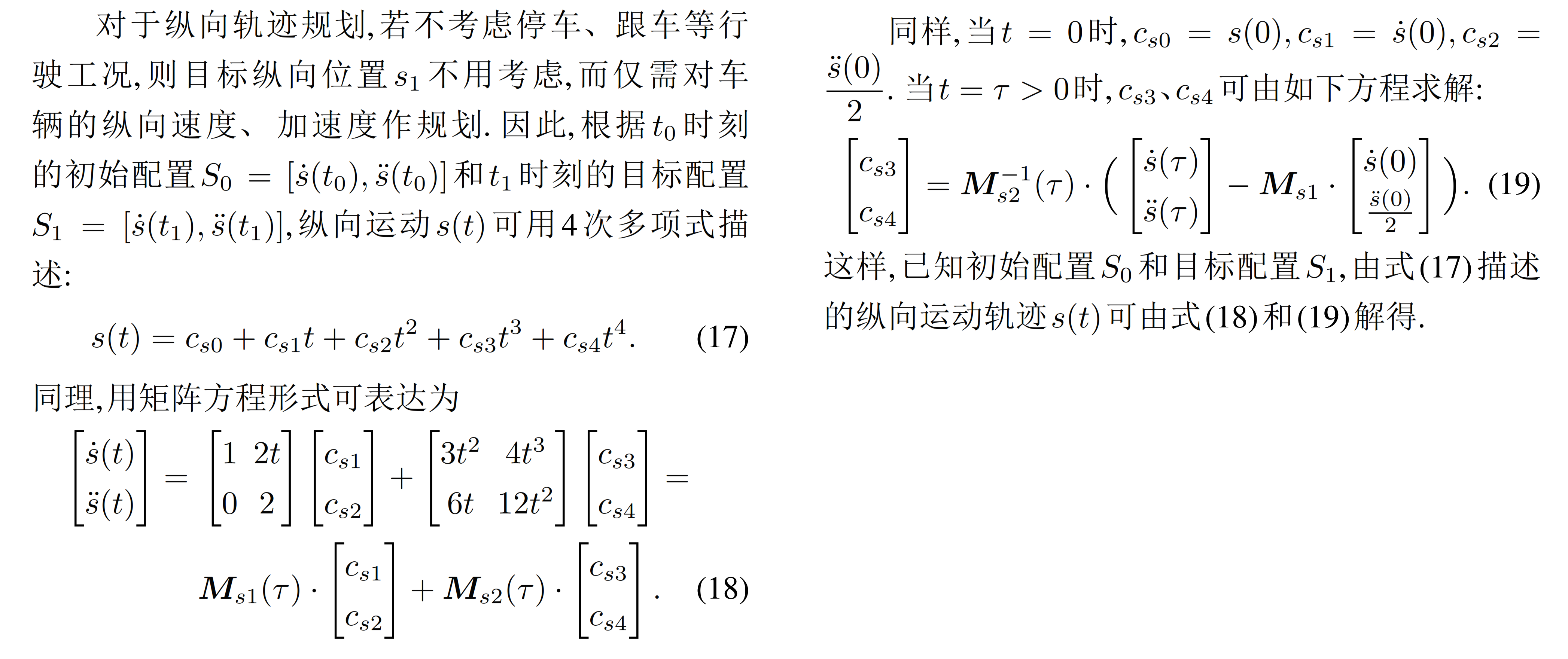三、 轨迹规划与最优选择

注意：
在实际编码过程中，基于Jerk最小化的损失函数中的积分计算通过分割采样取点，将积分转换成黎曼和的形式进行计算，具体原理如下：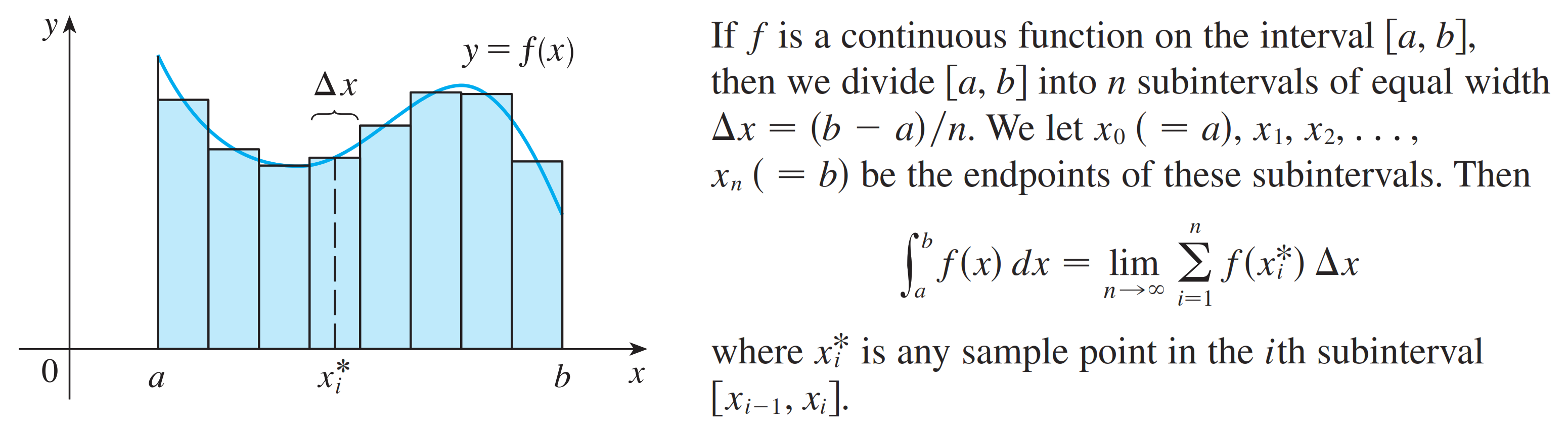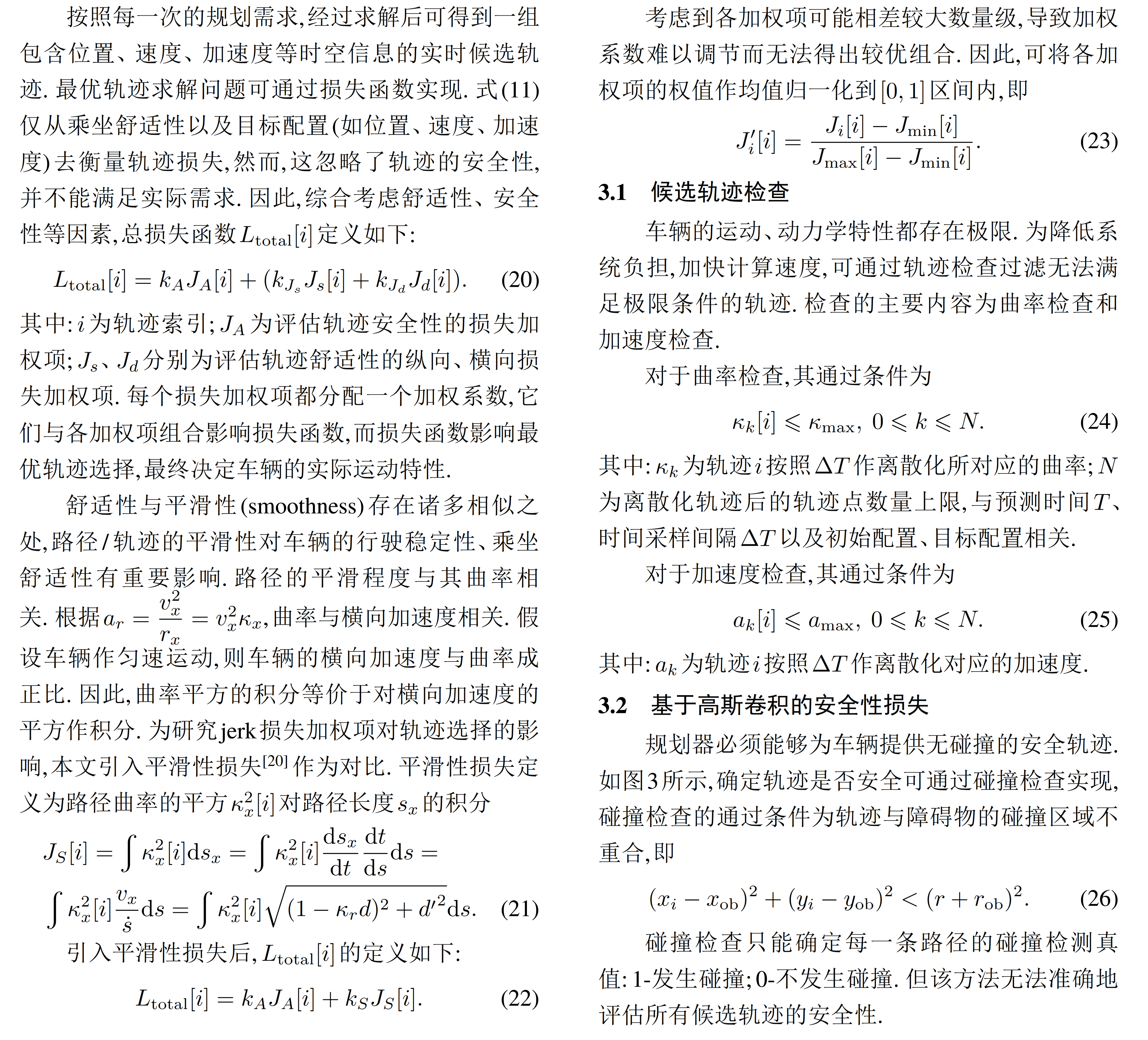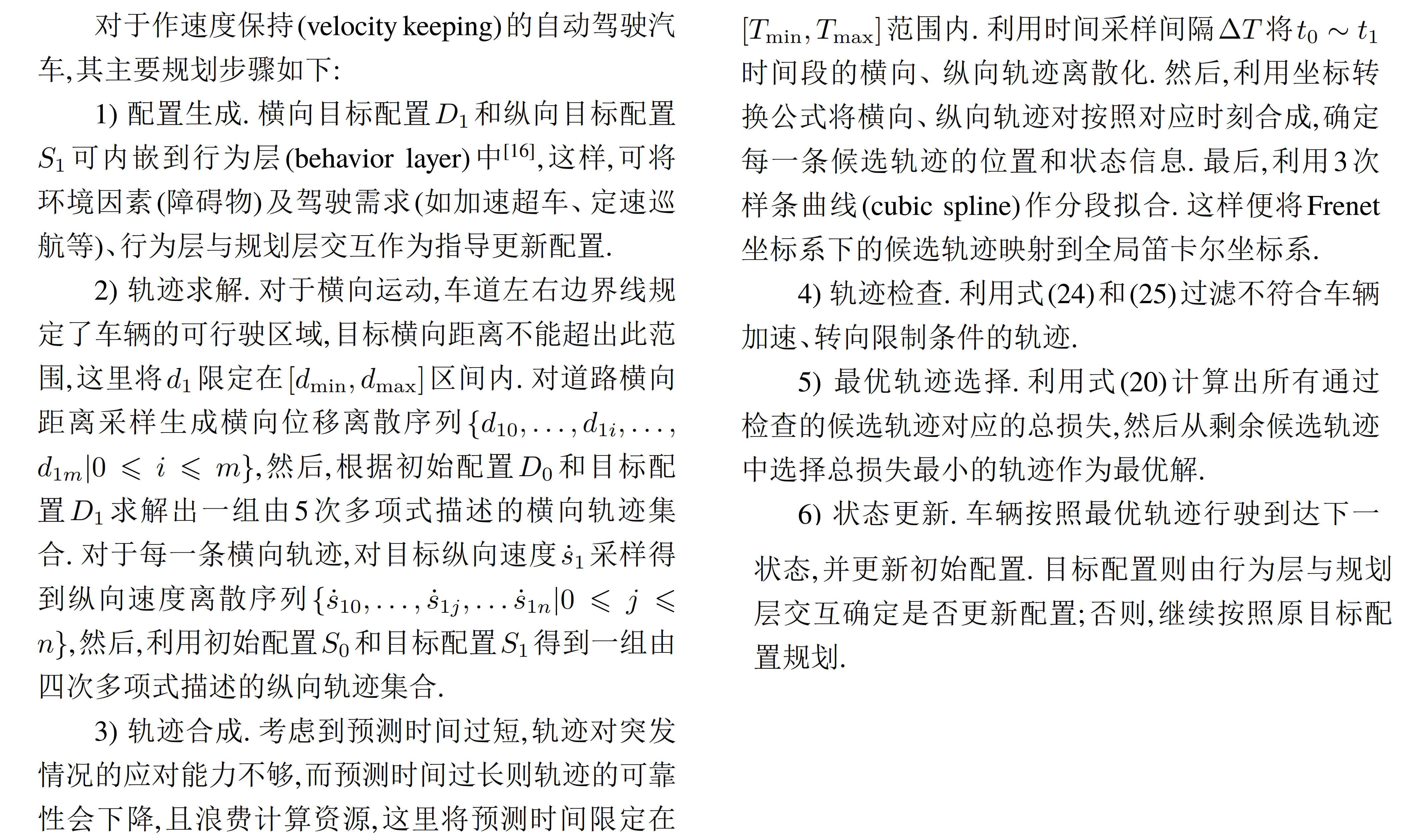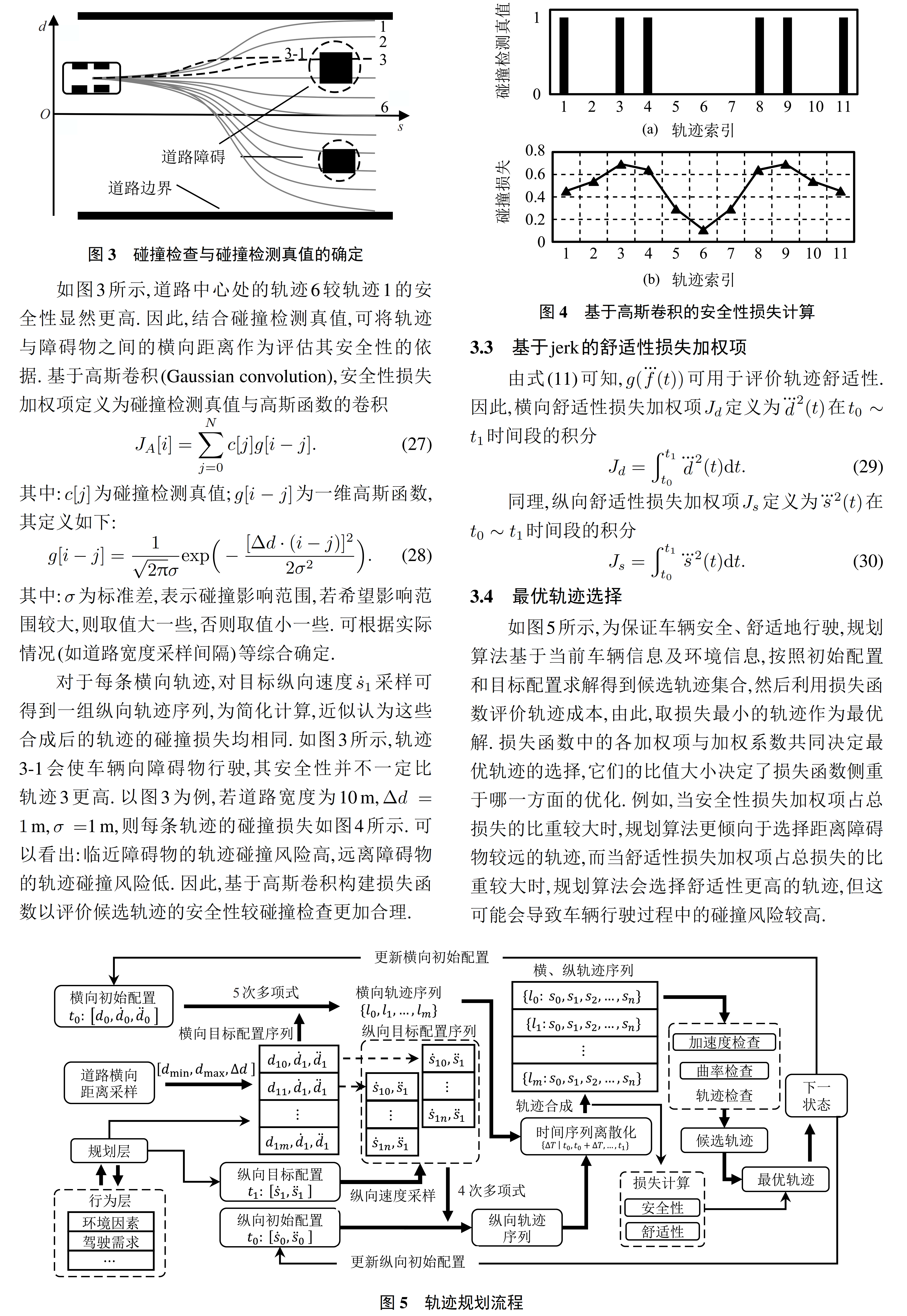四、 仿真评价与分析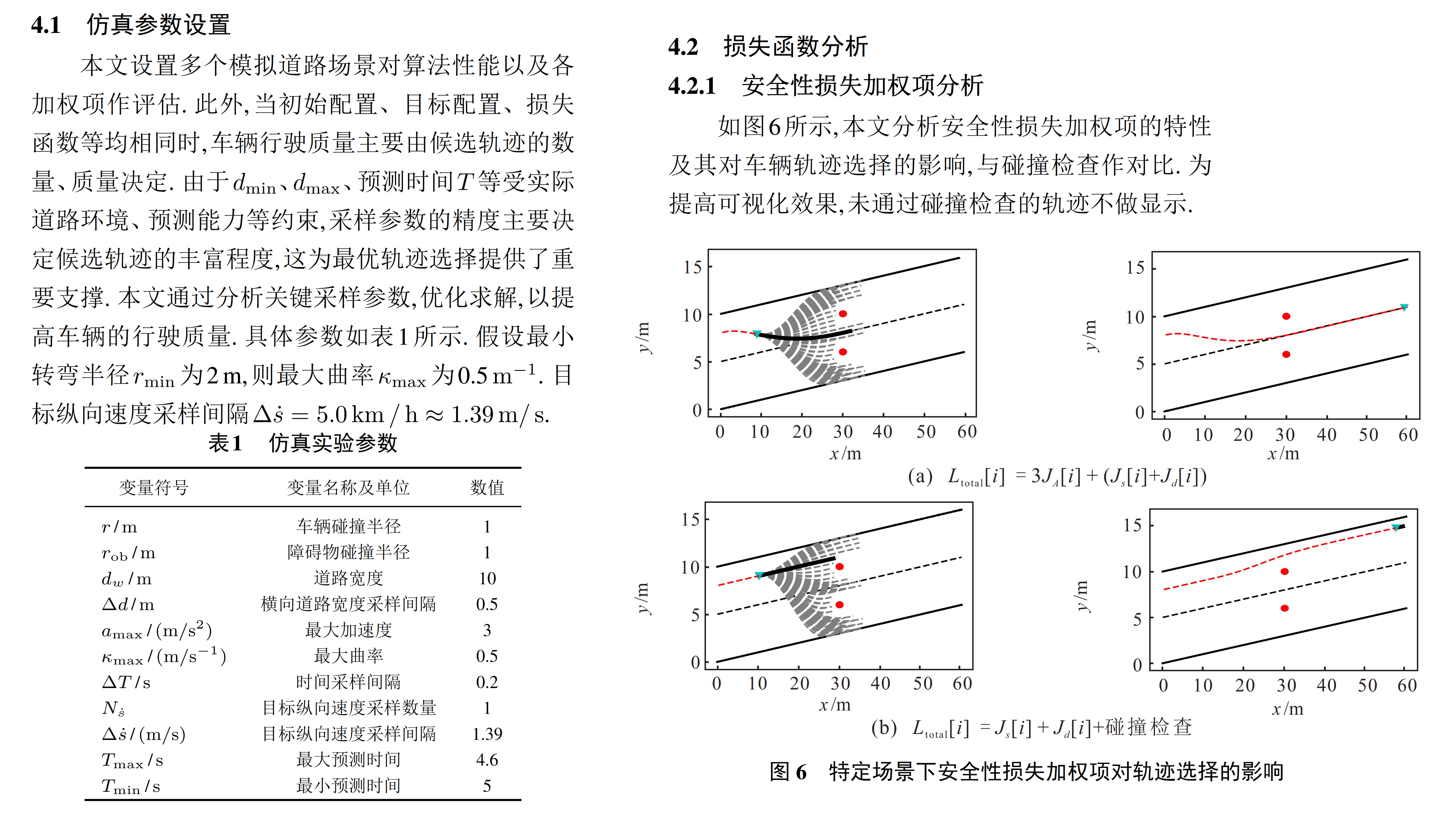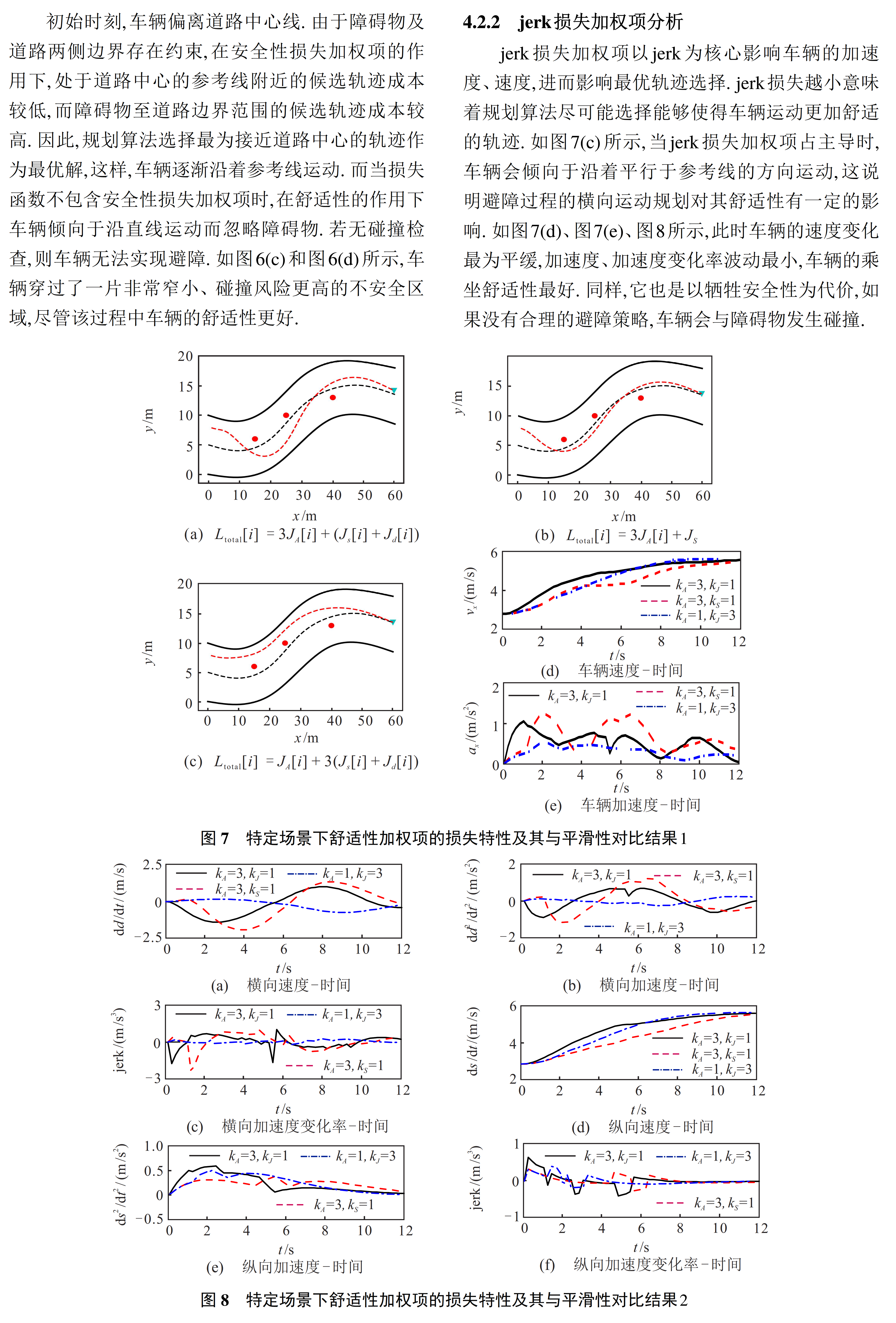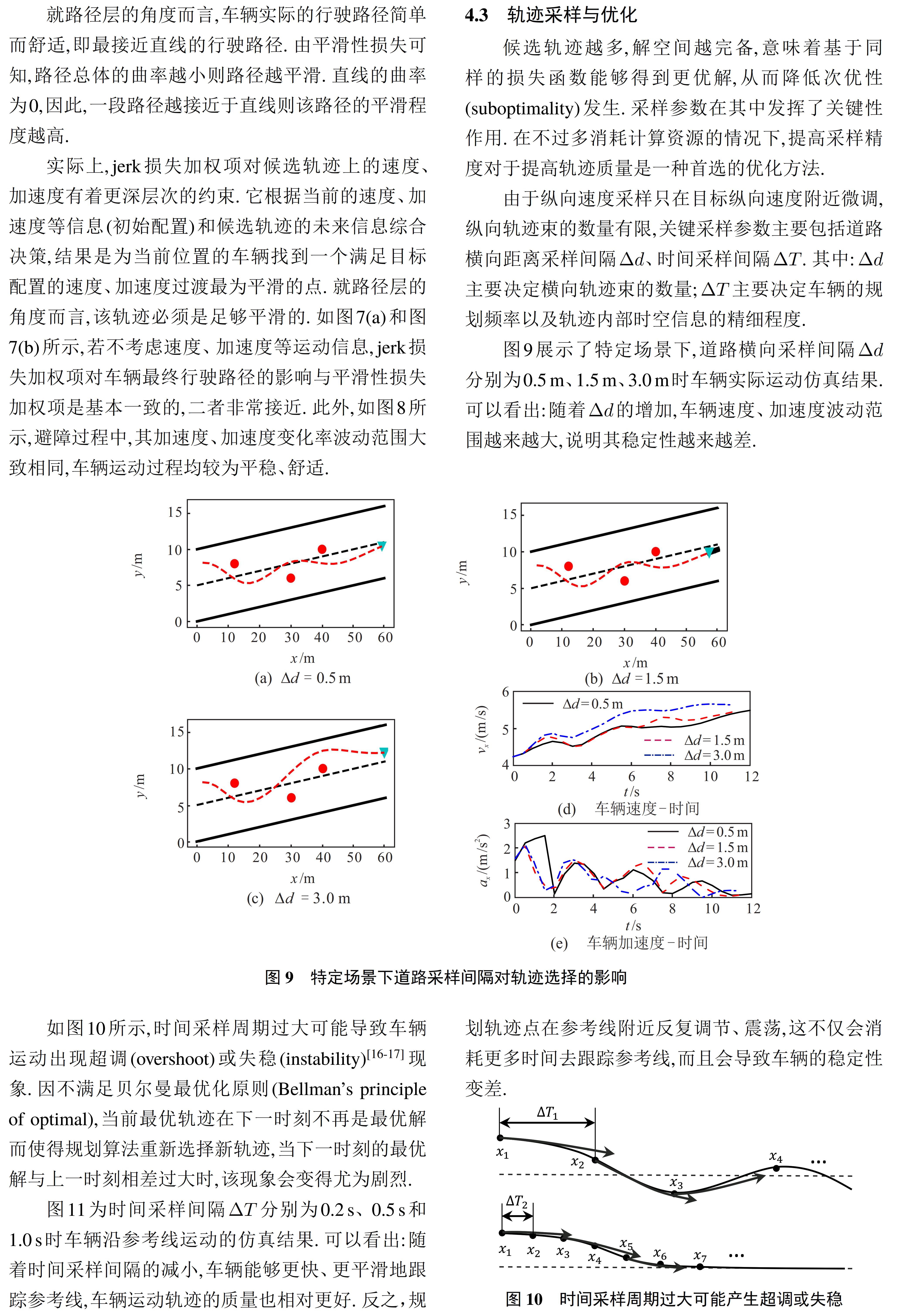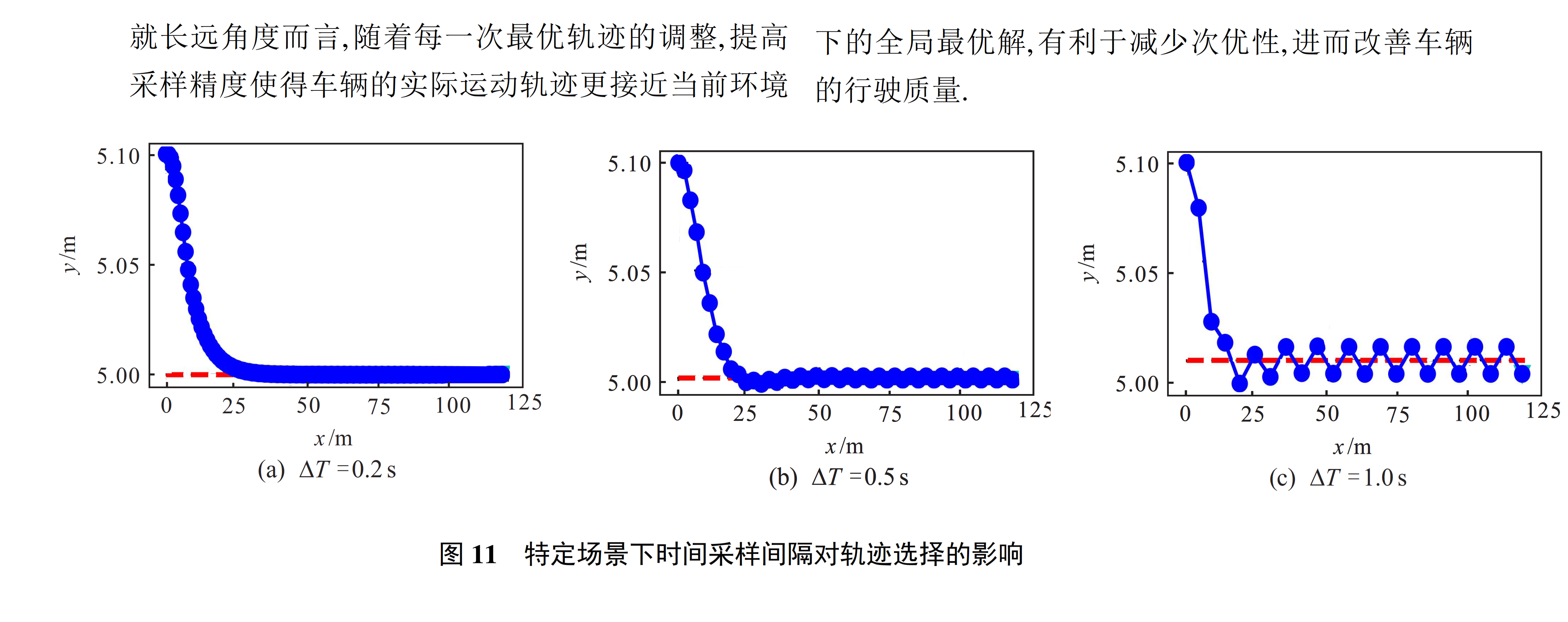五、Distance Pattern行驶工况(包括速度保持、距离保持)下基于场景选择的轨迹生成

参见论文：《Trajectory optimization and state selection for urban automated driving》2018

基于模式选择的轨迹生成流程

各工况说明

六、深入学习
《Robotics-Path-Planning-04-Quintic-Polynomial-Solver》Github
《硕士论文-基于Frenet坐标系采样的自动驾驶轨迹规划算法研究》
《Optimal Trajectory Generation for Dynamic Street Scenarios in a Frene´t Frame》
《无人驾驶汽车系统入门（二十一）——基于Frenet优化轨迹的无人车动作规划方法》
《Apollo项目坐标系研究》
《第三期 预测——Frenet 坐标》
维基百科：Frenet–Serret formulas

七、代码学习
Trajectory Planning in the Frenet Space
------基于论文《Optimal Trajectory Generation for Dynamic Street Scenarios in a Frene´t Frame》链接：Trajectory Planning in the Frenet Space - fjp.github.io
代码
'''
https://fjp.at/posts/optimal-frenet/
'''
import pdb

import time
import pylab as pl
from IPython import display

import matplotlib
import numpy as np
import matplotlib.pyplot as plt

import copy
import math

from cubic_spline_planner import *

class quintic_polynomial:
def __init__(self, xs, vxs, axs, xe, vxe, axe, T):
# calc coefficient of quintic polynomial
self.xs = xs
self.vxs = vxs
self.axs = axs
self.xe = xe
self.vxe = vxe
self.axe = axe

self.a0 = xs
self.a1 = vxs
self.a2 = axs / 2.0

A = np.array([[T ** 3, T ** 4, T ** 5],
[3 * T ** 2, 4 * T ** 3, 5 * T ** 4],
[6 * T, 12 * T ** 2, 20 * T ** 3]])
b = np.array([xe - self.a0 - self.a1 * T - self.a2 * T ** 2,
vxe - self.a1 - 2 * self.a2 * T,
axe - 2 * self.a2])
x = np.linalg.solve(A, b)

self.a3 = x
self.a4 = x
self.a5 = x

def calc_point(self, t):
xt = self.a0 + self.a1 * t + self.a2 * t ** 2 + \
self.a3 * t ** 3 + self.a4 * t ** 4 + self.a5 * t ** 5

return xt

def calc_first_derivative(self, t):
xt = self.a1 + 2 * self.a2 * t + \
3 * self.a3 * t ** 2 + 4 * self.a4 * t ** 3 + 5 * self.a5 * t ** 4

return xt

def calc_second_derivative(self, t):
xt = 2 * self.a2 + 6 * self.a3 * t + 12 * self.a4 * t ** 2 + 20 * self.a5 * t ** 3

return xt

def calc_third_derivative(self, t):
xt = 6 * self.a3 + 24 * self.a4 * t + 60 * self.a5 * t ** 2

return xt

class quartic_polynomial:
def __init__(self, xs, vxs, axs, vxe, axe, T):
# calc coefficient of quintic polynomial
self.xs = xs
self.vxs = vxs
self.axs = axs
self.vxe = vxe
self.axe = axe

self.a0 = xs
self.a1 = vxs
self.a2 = axs / 2.0

A = np.array([[3 * T ** 2, 4 * T ** 3],
[6 * T, 12 * T ** 2]])
b = np.array([vxe - self.a1 - 2 * self.a2 * T,
axe - 2 * self.a2])
x = np.linalg.solve(A, b)

self.a3 = x
self.a4 = x

def calc_point(self, t):
xt = self.a0 + self.a1 * t + self.a2 * t ** 2 + \
self.a3 * t ** 3 + self.a4 * t ** 4

return xt

def calc_first_derivative(self, t):
xt = self.a1 + 2 * self.a2 * t + \
3 * self.a3 * t ** 2 + 4 * self.a4 * t ** 3

return xt

def calc_second_derivative(self, t):
xt = 2 * self.a2 + 6 * self.a3 * t + 12 * self.a4 * t ** 2

return xt

def calc_third_derivative(self, t):
xt = 6 * self.a3 + 24 * self.a4 * t

return xt

class Frenet_path:
def __init__(self):
self.t = []
self.d = []
self.d_d = []
self.d_dd = []
self.d_ddd = []
self.s = []
self.s_d = []
self.s_dd = []
self.s_ddd = []
self.cd = 0.0
self.cv = 0.0
self.cf = 0.0

self.x = []
self.y = []
self.yaw = []
self.ds = []
self.c = []

# Parameter
MAX_SPEED = 50.0 / 3.6  # maximum speed [m/s]
MAX_ACCEL = 2.0  # maximum acceleration [m/ss]
MAX_CURVATURE = 1.0  # maximum curvature [1/m]
DT = 0.2  # time tick [s]
MAXT = 5.0  # max prediction time [s]
MINT = 4.0  # min prediction time [s]
TARGET_SPEED = 30.0 / 3.6  # target speed [m/s]
D_T_S = 5.0 / 3.6  # target speed sampling length [m/s]
N_S_SAMPLE = 1  # sampling number of target speed

# cost weights
KJ = 0.1
KT = 0.1
KD = 1.0
KLAT = 1.0
KLON = 1.0

def calc_frenet_paths(c_speed, c_d, c_d_d, c_d_dd, s0):
frenet_paths = []

# generate path to each offset goal

# Lateral motion planning
for Ti in np.arange(MINT, MAXT, DT):
print('di={0},Ti={1}'.format(di,Ti))
fp = Frenet_path()

lat_qp = quintic_polynomial(c_d, c_d_d, c_d_dd, di, 0.0, 0.0, Ti)

fp.t = [t for t in np.arange(0.0, Ti, DT)] //通过取样分割，便于后面将0-Ti的积分转换为黎曼和（即每个分割点处的值之和）
fp.d = [lat_qp.calc_point(t) for t in fp.t]
fp.d_d = [lat_qp.calc_first_derivative(t) for t in fp.t]
fp.d_dd = [lat_qp.calc_second_derivative(t) for t in fp.t]
fp.d_ddd = [lat_qp.calc_third_derivative(t) for t in fp.t]

# Loongitudinal motion planning (Velocity keeping)
for tv in np.arange(TARGET_SPEED - D_T_S * N_S_SAMPLE, TARGET_SPEED + D_T_S * N_S_SAMPLE, D_T_S):
tfp = copy.deepcopy(fp) #not tfp=fp

lon_qp = quartic_polynomial(s0, c_speed, 0.0, tv, 0.0, Ti)

tfp.s = [lon_qp.calc_point(t) for t in fp.t]
tfp.s_d = [lon_qp.calc_first_derivative(t) for t in fp.t]
tfp.s_dd = [lon_qp.calc_second_derivative(t) for t in fp.t]
tfp.s_ddd = [lon_qp.calc_third_derivative(t) for t in fp.t]

Jp = sum(np.power(tfp.d_ddd, 2))  # square of jerk ,the set T is discretized, and the integral is replaced with a summation
Js = sum(np.power(tfp.s_ddd, 2))  # square of jerk,the set T is discretized, and the integral is replaced with a summation

# square of diff from target speed
ds = (TARGET_SPEED - tfp.s_d[-1]) ** 2

tfp.cd = KJ * Jp + KT * Ti + KD * tfp.d[-1] ** 2
tfp.cv = KJ * Js + KT * Ti + KD * ds
tfp.cf = KLAT * tfp.cd + KLON * tfp.cv

frenet_paths.append(tfp)

return frenet_paths

faTrajX = []
faTrajY = []

def calc_global_paths(fplist, csp):
# faTrajX = []
# faTrajY = []

for fp in fplist:

# calc global positions
for i in range(len(fp.s)):
ix, iy = csp.calc_position(fp.s[i])
if ix is None:
break
iyaw = csp.calc_yaw(fp.s[i])
di = fp.d[i]
fx = ix + di * math.cos(iyaw + math.pi / 2.0)
fy = iy + di * math.sin(iyaw + math.pi / 2.0)
fp.x.append(fx)
fp.y.append(fy)

# Just for plotting
faTrajX.append(fp.x)
faTrajY.append(fp.y)

# calc yaw and ds
for i in range(len(fp.x) - 1):
dx = fp.x[i + 1] - fp.x[i]
dy = fp.y[i + 1] - fp.y[i]
fp.yaw.append(math.atan2(dy, dx))
fp.ds.append(math.sqrt(dx ** 2 + dy ** 2))

fp.yaw.append(fp.yaw[-1])
fp.ds.append(fp.ds[-1])

# calc curvature
for i in range(len(fp.yaw) - 1):
fp.c.append((fp.yaw[i + 1] - fp.yaw[i]) / fp.ds[i])

return fplist

faTrajCollisionX = []
faTrajCollisionY = []
faObCollisionX = []
faObCollisionY = []

def check_collision(fp, ob):
# pdb.set_trace()
for i in range(len(ob[:, 0])):
# Calculate the distance for each trajectory point to the current object
d = [((ix - ob[i, 0]) ** 2 + (iy - ob[i, 1]) ** 2)
for (ix, iy) in zip(fp.x, fp.y)]

# Check if any trajectory point is too close to the object using the robot radius
collision = any([di <= ROBOT_RADIUS ** 2 for di in d])

if collision:
# plot(ft.x, ft.y, 'rx')
faTrajCollisionX.append(fp.x)
faTrajCollisionY.append(fp.y)

# plot(ox, oy, 'yo');
# pdb.set_trace()
if ob[i, 0] not in faObCollisionX or ob[i, 1] not in faObCollisionY:
faObCollisionX.append(ob[i, 0])
faObCollisionY.append(ob[i, 1])

return True

return False

# faTrajOkX = []
# faTrajOkY = []

def check_paths(fplist, ob):
okind = []
for i in range(len(fplist)):
if any([v > MAX_SPEED for v in fplist[i].s_d]):  # Max speed check
continue
elif any([abs(a) > MAX_ACCEL for a in fplist[i].s_dd]):  # Max accel check
continue
elif any([abs(c) > MAX_CURVATURE for c in fplist[i].c]):  # Max curvature check
continue
elif check_collision(fplist[i], ob):
continue

okind.append(i)

return [fplist[i] for i in okind]

fpplist = []

def frenet_optimal_planning(csp, s0, c_speed, c_d, c_d_d, c_d_dd, ob):
# pdb.set_trace()
fplist = calc_frenet_paths(c_speed, c_d, c_d_d, c_d_dd, s0)
fplist = calc_global_paths(fplist, csp)
fplist = check_paths(fplist, ob)

# fpplist = deepcopy(fplist)
fpplist.extend(fplist)

# find minimum cost path
mincost = float("inf")
bestpath = None
for fp in fplist:
if mincost >= fp.cf:
mincost = fp.cf
bestpath = fp

return bestpath

from cubic_spline_planner import *

def generate_target_course(x, y):
csp = Spline2D(x, y)
s = np.arange(0, csp.s[-1], 0.1)

rx, ry, ryaw, rk = [], [], [], []
for i_s in s:
ix, iy = csp.calc_position(i_s)
rx.append(ix)
ry.append(iy)
ryaw.append(csp.calc_yaw(i_s))
rk.append(csp.calc_curvature(i_s))

return rx, ry, ryaw, rk, csp

show_animation = True
# show_animation = False
# way points
wx = [0.0, 10.0, 20.5, 35.0, 70.5]
wy = [0.0, -6.0, 5.0, 6.5, 0.0]
# obstacle lists
ob = np.array([[20.0, 10.0],
[30.0, 6.0],
[30.0, 8.0],
[35.0, 8.0],
[50.0, 3.0]
])

tx, ty, tyaw, tc, csp = generate_target_course(wx, wy)

# initial state
c_speed = 10.0 / 3.6  # current speed [m/s]
c_d = 2.0  # current lateral position [m]
c_d_d = 0.0  # current lateral speed [m/s]
c_d_dd = 0.0  # current latral acceleration [m/s]
s0 = 0.0  # current course position

area = 20.0  # animation area length [m]

fig = plt.figure()
plt.ion()

faTx = tx
faTy = ty
faObx = ob[:, 0]
faOby = ob[:, 1]
faPathx = []
faPathy = []
faRobotx = []
faRoboty = []
faSpeed = []
for i in range(100):
path = frenet_optimal_planning(csp, s0, c_speed, c_d, c_d_d, c_d_dd, ob)
s0 = path.s
c_d = path.d
c_d_d = path.d_d
c_d_dd = path.d_dd
c_speed = path.s_d

if np.hypot(path.x - tx[-1], path.y - ty[-1]) <= 1.0:
print("Goal")
break

faPathx.append(path.x[1:])
faPathy.append(path.y[1:])
faRobotx.append(path.x)
faRoboty.append(path.y)
faSpeed.append(c_speed)
if show_animation:
plt.cla()
plt.plot(tx, ty, animated=True)
plt.plot(ob[:, 0], ob[:, 1], "xk")
plt.plot(tx,ty,'-',color='crimson')

plt.plot(path.x, path.y, "vc")

for (ix, iy) in zip(faTrajX, faTrajY):
# pdb.set_trace()
plt.plot(ix[1:], iy[1:], '-', color=[0.5, 0.5, 0.5])

faTrajX = []
faTrajY = []

for (ix, iy) in zip(faTrajCollisionX, faTrajCollisionY):
# pdb.set_trace()
plt.plot(ix[1:], iy[1:], 'rx')
faTrajCollisionX = []
faTrajCollisionY = []
# pdb.set_trace()
for fp in fpplist:
# pdb.set_trace()
plt.plot(fp.x[1:], fp.y[1:], '-g')
fpplist = []

# pdb.set_trace()
for (ix, iy) in zip(faObCollisionX, faObCollisionY):
# pdb.set_trace()
plt.plot(ix, iy, 'oy')
faObCollisionX = []
faObCollisionY = []

plt.plot(path.x[1:], path.y[1:], "-ob")
print('len:{}'.format(len(path.x[1:])))

plt.xlim(path.x - area, path.x[-1] + area)
plt.ylim(path.y - area, path.y[-1] + area)
plt.title("v[km/h]:" + str(c_speed * 3.6)[0:4])
plt.grid(True)
plt.pause(0.00001)
plt.show()
# display.clear_output(wait=True)
# display.display(pl.gcf())
plt.pause(0.1)
print("Finish")

plt.ioff()


运行结果（片段）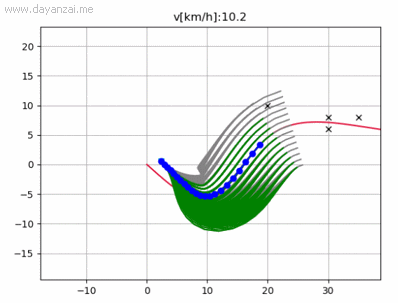展开全文ubuntu linux 自动驾驶
• ## Frenet坐标系与Cartesian坐标系互转

千次阅读 多人点赞 2020-09-23 20:35:13
Frenet坐标系在无人驾驶领域被普遍使用，特别是在城市、高速等道路交通环境下无人驾驶的路径规划系统中。Frenet坐标系使用道路的中心线作为Base frame，使用参考线的切线向量和法线向量建立坐标系。相比笛卡尔坐标系...

Frenet坐标系在无人驾驶领域被普遍使用，特别是在城市、高速等道路交通环境下无人驾驶的路径规划系统中。Frenet坐标系使用道路的中心线作为Base frame，使用参考线的切线向量和法线向量建立坐标系。相比笛卡尔坐标系，Frenet坐标系简化了路径规划问题。网上关于Frenet坐标系的博客或资料很多，此处不再赘述。本文先给出简单的转换公式推导过程，然后给出三对Frenet坐标系与Cartesian坐标系互转的python代码与使用示例方便自己查阅使用。详细推导参见博客Apollo项目坐标系研究。note：下图中Frenet的q坐标轴也可以用d或l表示。文章目录
一、 Frenet坐标系与Cartesian坐标系的转换公式简单推导1.1 Frenet公式1.2 简单推导过程已知

x

⃗

=

[

x

x

,

y

x

]

\vec{x}=[x_{x}, y_{x}]

推导

[

s

,

l

]

[s, l]

已知

x

⃗

=

[

x

x

,

y

x

]

,

θ

x

,

v

x

\vec{x}=[x_{x}, y_{x}],\theta_{x},v_x

推导

[

s

,

l

,

s

˙

,

l

′

]

[s, l,\dot{s},l']

已知

x

⃗

=

[

x

x

,

y

x

]

,

θ

x

,

v

x

,

a

x

,

k

x

\vec{x}=[x_{x}, y_{x}],\theta_{x},v_x,a_x,k_{x}

推导

[

s

,

l

,

s

˙

,

l

′

,

s

¨

,

l

′

′

]

[s, l,\dot{s},l',\ddot{s},l'']

综合以上可得转换公式

二、Python代码函数实现1D

[

x

,

y

]

→

[

s

,

d

]

[x, y]\rightarrow[s, d]

1D

[

s

,

d

]

→

[

x

,

y

]

[s, d]\rightarrow[x, y]

2D

[

x

,

y

,

v

,

θ

]

→

[

s

,

s

˙

,

d

,

d

′

]

[x, y, v, \theta]\rightarrow [s, \dot{s}, d,d']

2D

[

s

,

s

˙

,

d

,

d

′

]

→

[

x

,

y

,

v

,

θ

]

[s, \dot{s}, d,d']\rightarrow [x, y, v, \theta]

3D

[

x

,

y

,

v

,

θ

,

k

,

a

]

→

[

s

,

s

˙

,

s

¨

,

d

,

d

′

,

d

′

′

]

[x, y, v, \theta, k,a]\rightarrow [s, \dot{s},\ddot{s}, d,d',d'']

3D

[

s

,

s

˙

,

s

¨

,

d

,

d

′

,

d

′

′

]

→

[

x

,

y

,

v

,

θ

,

k

,

a

]

[s, \dot{s},\ddot{s}, d,d',d'']\rightarrow [x, y, v, \theta, k,a]

三、应用示例例1. 现在我知道车道的宽度为

3.0

3.0

，利用frenet_to_cartesian1D获取车道的边界线：例2. 已知道路中有一个障碍物的Cartesian坐标为

(

2.0

,

3.5

)

(2.0, 3.5)

，求它以道路中心线为base frame的Frenet坐标例3. 在Frenet坐标系下生成一簇候选轨迹，将其转换到Cartesian坐标例4. 如果想要知道候选轨迹上各点的角度、曲率等信息，首先得计算出base frame曲线的曲率与曲率导数等信息补充CalculateKappa(rkappa, rdkappa, l, dl, ddl)中求曲率的公式

一、 Frenet坐标系与Cartesian坐标系的转换公式简单推导
1.1 Frenet公式
下图显示了一条3D空间中一条连续可微的曲线K，P为曲线K上的一个点，黄色平面为曲线K在点P处的运动平面。

T

⃗

\vec{T}

为曲线K在点P处的切向量，

N

⃗

\vec{N}

为K在P处的法向量（

N

⃗

\vec{N}

与

T

⃗

\vec{T}

在同一运动平面）,

B

⃗

\vec{B}

为曲线K在P处的副法向量（

N

⃗

\vec{N}

垂直于运动平面）。有Frenet公式：

{

d

T

⃗

d

s

=

k

N

⃗

d

N

⃗

d

s

=

−

k

T

⃗

+

τ

B

⃗

d

B

⃗

d

s

=

−

τ

N

⃗

\left\{\begin{matrix} \frac{d\vec{T}}{ds}= & k\vec{N} \\ \frac{d\vec{N}}{ds}= & -k\vec{T}+\tau\vec{B} \\ \frac{d\vec{B}}{ds}= & -\tau\vec{N} \end{matrix}\right.

其中，

d

d

s

\frac{d}{ds}

表示某一方向向量对弧长

s

s

的导数，

k

k

为曲率（ curvature），

τ

\tau

为挠率（ torsion）。
曲率：曲线不能形成一条直线的度量值，曲率越趋于0，则曲线越趋近于直线；挠率：是曲线不能形成在同一平面内运动曲线的度量值，挠率越趋于0，则曲线越趋近于在同一平面内运动。
在大地表面，局部路面可看作一个平面，对于无人驾驶路径规划应用，挠率可设定为0。从而Frenet公式进一步简化为：

{

d

T

⃗

d

s

=

k

N

⃗

d

N

⃗

d

s

=

−

k

T

⃗

(1)

\left\{\begin{matrix} \frac{d\vec{T}}{ds}= & k\vec{N} \\ \frac{d\vec{N}}{ds}= & -k\vec{T} \end{matrix}\right. \tag{1}

1.2 简单推导过程
引博客Apollo项目坐标系研究中的图如下：Frenet坐标系：

[

s

,

s

˙

,

s

¨

,

l

,

l

˙

,

l

¨

,

l

′

,

l

′

′

]

[s, \dot{s}, \ddot{s}, l, \dot{l}, \ddot{l}, l', l'']

，Cartesian坐标系：

[

x

⃗

,

v

x

,

a

a

,

θ

x

,

k

x

]

[\vec{x},v_{x}, a_{a}, \theta_{x},k_{x}]

，释义如下：

s

s

：Frenet纵坐标；

s

˙

=

d

s

d

t

\dot{s}=\frac{ds}{dt}

：Frenet纵坐标对时间的导数，也即沿base frame的速度；

s

¨

=

d

s

˙

d

t

\ddot{s}=\frac{d\dot{s}}{dt}

：沿base frame的加速度；

l

l

：Frenet横坐标；

l

˙

=

d

l

d

t

\dot{l}=\frac{dl}{dt}

：Frenet横向速度；

l

¨

=

d

l

˙

d

t

\ddot{l}=\frac{d\dot{l}}{dt}

：Frenet横向加速度；

l

′

=

d

l

d

s

l'=\frac{dl}{ds}

：Frenet横向坐标对纵向坐标的导数；

l

′

′

=

d

l

′

d

s

l''=\frac{dl'}{ds}

：Frenet横向坐标对纵向坐标的二阶导；

x

⃗

\vec{x}

：为对应Cartesian坐标系下的坐标，是一个向量；

θ

x

\theta_{x}

：Cartesian坐标系下的朝向；

k

x

=

d

θ

x

d

s

k_{x}=\frac{d\theta_{x}}{ds}

：曲率；

v

x

=

∣

∣

x

⃗

˙

∣

∣

2

v_{x}=||\dot{\vec{x}}||_{2}

：Cartesian坐标系下的线速度；

a

x

=

d

v

x

d

t

a_{x}=\frac{dv_{x}}{dt}

：Cartesian坐标系下的加速度；
已知

x

⃗

=

[

x

x

,

y

x

]

\vec{x}=[x_{x}, y_{x}]

推导

[

s

,

l

]

[s, l]

求

s

s

道先，找到曲线上离

[

x

x

,

y

x

]

[x_{x},y_{x}]

最近的参考点

r

⃗

=

[

x

r

,

y

r

]

\vec{r}=[x_{r}, y_{r}]

，该参考点处的

s

s

即为

[

x

x

,

y

x

]

[x_{x}, y_{x}]

在Frenet坐标系下的

s

s

。  求

l

l

x

⃗

,

r

⃗

\vec{x},\vec{r}

分别为参考点与待转换点在Cartesian坐标系下的向量，我们有

x

⃗

=

r

⃗

+

l

N

r

⃗

\vec{x}=\vec{r}+l\vec{N_{r}}

，变换可得：

l

=

(

x

⃗

−

r

⃗

)

T

N

r

⃗

=

∣

∣

x

⃗

−

r

⃗

∣

∣

2

cos

⁡

(

θ

x

−

r

−

(

θ

r

+

π

2

)

)

=

∣

∣

x

⃗

−

r

⃗

∣

∣

2

(

sin

⁡

(

θ

x

−

r

)

cos

⁡

(

θ

r

)

−

cos

⁡

(

θ

x

−

r

)

sin

⁡

(

θ

r

)

)

l=(\vec{x}-\vec{r})^{T}\vec{N_{r}}=||\vec{x}-\vec{r}||_{2}\cos(\theta_{x-r}-(\theta_{r}+\frac{\pi}{2}))=||\vec{x}-\vec{r}||_{2}(\sin(\theta_{x-r})\cos(\theta_{r})-\cos(\theta_{x-r})\sin(\theta_{r}))

备注：上式中，

θ

x

−

r

\theta_{x-r}

为向量

x

⃗

−

r

⃗

\vec{x}-\vec{r}

的方向角度，

θ

r

+

π

2

\theta_{r}+\frac{\pi}{2}

为单位向量

N

⃗

r

\vec{N}_{r}

的方向角度（对比上一张图可知），则上式可利用向量点乘公式

a

⃗

×

b

⃗

=

∣

a

∣

∣

b

∣

cos

⁡

θ

\vec{a}\times \vec{b}=|a||b|\cos{\theta}

得到。此处，

θ

\theta

为向量

a

⃗

\vec{a}

与向量

b

⃗

\vec{b}

之间的夹角。

假定

x

⃗

=

(

x

x

,

y

x

)

,

r

⃗

=

(

x

r

,

y

r

)

\vec{x}=(x_{x}, y_{x}), \vec{r}=(x_{r}, y_{r})

，则

∣

∣

x

⃗

−

r

⃗

∣

∣

2

=

(

x

x

−

x

r

)

2

+

(

y

x

−

y

r

)

2

||\vec{x}-\vec{r}||_{2}=\sqrt{(x_{x}-x_{r})^2+(y_{x}-y_{r})^2}

在Frenet坐标系下，每个点到base frame上参考点的向量都与该参考点的法向量

N

⃗

\vec{N}

同向或反向，因此，

sin

⁡

(

θ

x

−

r

)

cos

⁡

(

θ

r

)

−

cos

⁡

(

θ

x

−

r

)

sin

⁡

(

θ

r

)

=

1

或

−

1

\sin(\theta_{x-r})\cos(\theta_{r})-\cos(\theta_{x-r})\sin(\theta_{r})=1 或 -1

。

θ

x

−

r

\theta_{x-r}

为向量

x

⃗

−

r

⃗

\vec{x}-\vec{r}

的角度，因此，

sin

⁡

(

θ

x

−

r

)

cos

⁡

(

θ

x

−

r

)

=

y

x

−

y

r

x

x

−

x

r

\frac{\sin(\theta_{x-r})}{\cos(\theta_{x-r})}=\frac{y_{x}-y_{r}}{x_{x}-x_{r}}

。可以根据

(

x

x

−

x

r

)

2

+

(

y

x

−

y

r

)

2

\sqrt{(x_{x}-x_{r})^2+(y_{x}-y_{r})^2}

来确定

l

l

的绝对取值，可根据

(

y

x

−

y

r

)

cos

⁡

(

θ

r

)

−

(

x

x

−

x

r

)

sin

⁡

(

θ

r

)

(y_{x}-y_{r})\cos(\theta_{r})-(x_{x}-x_{r})\sin(\theta_{r})

的正负来确定

l

l

的正负号，公式如下：

l

=

s

i

g

n

(

(

y

x

−

y

r

)

cos

⁡

(

θ

r

)

−

(

x

x

−

x

r

)

sin

⁡

(

θ

r

)

)

(

x

x

−

x

r

)

2

+

(

y

x

−

y

r

)

2

(2)

l = sign\left((y_{x}-y_{r})\cos(\theta_{r})-(x_{x}-x_{r})\sin(\theta_{r})\right)\sqrt{(x_{x}-x_{r})^2+(y_{x}-y_{r})^2} \tag{2}

已知

x

⃗

=

[

x

x

,

y

x

]

,

θ

x

,

v

x

\vec{x}=[x_{x}, y_{x}],\theta_{x},v_x

推导

[

s

,

l

,

s

˙

,

l

′

]

[s, l,\dot{s},l']

求

l

′

l'

由

l

=

(

x

⃗

−

r

⃗

)

T

N

r

⃗

l=(\vec{x}-\vec{r})^{T}\vec{N_{r}}

可得:

l

˙

=

(

x

⃗

˙

−

r

⃗

˙

)

T

N

r

⃗

+

(

x

⃗

−

r

⃗

)

T

N

r

⃗

˙

=

v

x

T

x

⃗

T

N

r

⃗

−

s

˙

T

r

⃗

T

N

r

⃗

+

l

N

r

⃗

T

N

r

⃗

˙

\dot{l}=(\dot{\vec{x}}-\dot{\vec{r}})^{T}\vec{N_{r}}+(\vec{x}-\vec{r})^{T}\dot{\vec{N_{r}}} \\ = v_{x}\vec{T_{x}}^{T}\vec{N_{r}}-\dot{s}\vec{T_{r}}^{T}\vec{N_{r}}+l\vec{N_{r}}^{T}\dot{\vec{N_{r}}}

由Frenet公式（见上式式(1)）有

N

r

⃗

′

=

d

N

r

⃗

d

s

=

−

k

r

T

r

⃗

\vec{N_{r}}'=\frac{d\vec{N_{r}}}{ds}=-k_{r}\vec{T_{r}}

，于是有

N

r

⃗

˙

=

d

s

d

t

d

N

r

⃗

d

s

=

−

k

r

s

˙

T

r

⃗

\dot{\vec{N_{r}}}=\frac{ds}{dt}\frac{d\vec{N_{r}}}{ds}=-k_{r}\dot{s}\vec{T_{r}}

，代入上式可得：

l

˙

=

v

x

T

x

⃗

T

N

r

⃗

−

s

˙

T

r

⃗

T

N

r

⃗

−

l

s

˙

k

r

N

r

⃗

T

T

r

⃗

\dot{l}=v_{x}\vec{T_{x}}^{T}\vec{N_{r}}-\dot{s}\vec{T_{r}}^{T}\vec{N_{r}}-l\dot{s}k_{r}\vec{N_{r}}^{T}\vec{T_{r}}

由于

N

r

⃗

\vec{N_{r}}

与

T

r

⃗

\vec{T_{r}}

正交

⇒

N

r

⃗

T

T

r

⃗

=

T

r

⃗

T

N

r

⃗

=

0

\Rightarrow \vec{N_{r}}^{T}\vec{T_{r}}=\vec{T_{r}}^{T}\vec{N_{r}}=0

，

T

x

⃗

T

N

r

⃗

=

cos

⁡

(

θ

x

−

θ

r

−

π

2

)

=

sin

⁡

(

θ

x

−

θ

r

)

\vec{T_{x}}^{T}\vec{N_{r}}=\cos(\theta_{x}-\theta_{r}-\frac{\pi}{2})=\sin(\theta_{x}-\theta_{r})

,代入上式得：

l

˙

=

v

x

sin

⁡

(

θ

x

−

θ

r

)

(3)

\dot{l}=v_{x}\sin(\theta_{x}-\theta_{r}) \tag{3}

x

⃗

˙

=

d

x

⃗

d

t

=

r

⃗

+

l

N

r

⃗

d

t

=

r

⃗

˙

+

l

˙

N

r

⃗

+

l

N

r

⃗

˙

=

s

˙

T

r

⃗

+

l

˙

N

r

⃗

−

l

k

r

s

˙

T

r

⃗

=

s

˙

(

1

−

l

k

r

)

T

r

⃗

+

l

˙

N

r

⃗

\dot{\vec{x}}=\frac{d\vec{x}}{dt}=\frac{\vec{r}+l\vec{N_{r}}}{dt}=\dot{\vec{r}}+\dot{l}\vec{N_{r}}+l\dot{\vec{N_{r}}}=\dot{s}\vec{T_{r}}+\dot{l}\vec{N_{r}}-lk_{r}\dot{s}\vec{T_{r}}=\dot{s}(1-lk_{r})\vec{T_{r}}+\dot{l}\vec{N_{r}}

v

x

=

∣

∣

x

⃗

˙

∣

∣

2

=

x

⃗

˙

T

x

⃗

˙

=

[

s

˙

(

1

−

k

r

l

)

]

2

+

l

˙

2

v_{x}=||\dot{\vec{x}}||_{2}=\sqrt{\dot{\vec{x}}^{T}\dot{\vec{x}}}=\sqrt{[\dot{s}(1-k_{r}l)]^{2}+\dot{l}^{2}}

l

′

2

=

(

d

l

d

s

)

2

=

(

l

˙

s

˙

)

2

=

(

v

x

sin

⁡

(

θ

x

−

θ

r

)

s

˙

)

2

=

sin

⁡

2

(

θ

x

−

θ

r

)

[

s

˙

(

1

−

k

r

l

)

]

2

+

l

˙

2

s

˙

2

=

sin

⁡

2

(

θ

x

−

θ

r

)

[

(

1

−

k

r

l

)

2

−

(

l

˙

s

˙

)

2

]

=

sin

⁡

2

(

θ

x

−

θ

r

)

[

(

1

−

k

r

l

)

2

−

l

′

2

]

⇒

l

′

=

(

1

−

k

r

l

)

tan

⁡

(

θ

x

−

θ

r

)

l'^{2}=(\frac{dl}{ds})^{2}=(\frac{\dot{l}}{\dot{s}})^{2}=(\frac{v_{x}\sin(\theta_{x}-\theta_{r})}{\dot{s}})^{2}=\sin^{2}(\theta_{x}-\theta_{r})\frac{[\dot{s}(1-k_{r}l)]^{2}+\dot{l}^{2}}{\dot{s}^{2}}=\sin^{2}(\theta_{x}-\theta_{r})[(1-k_{r}l)^{2}-(\frac{\dot{l}}{\dot{s}})^{2}]=\sin^{2}(\theta_{x}-\theta_{r})[(1-k_{r}l)^{2}-l'^{2}] \Rightarrow l'=(1-k_{r}l)\tan(\theta_{x}-\theta_{r})

，因此：

l

′

=

(

1

−

k

r

l

)

tan

⁡

(

θ

x

−

θ

r

)

(4)

l'=(1-k_{r}l)\tan(\theta_{x}-\theta_{r}) \tag{4}

求

s

˙

\dot{s}

由

l

′

=

v

x

sin

⁡

(

θ

x

−

θ

r

)

s

˙

l'=\frac{v_{x}\sin(\theta_{x}-\theta_{r})}{\dot{s}}

与

l

′

=

(

1

−

k

r

l

)

tan

⁡

(

θ

x

−

θ

r

)

l'=(1-k_{r}l)\tan(\theta_{x}-\theta_{r})

可得:

s

˙

=

v

x

cos

⁡

(

θ

x

−

θ

r

)

1

−

k

r

l

(5)

\dot{s}=\frac{v_{x}\cos(\theta_{x}-\theta_{r})}{1-k_{r}l}\tag{5}

已知

x

⃗

=

[

x

x

,

y

x

]

,

θ

x

,

v

x

,

a

x

,

k

x

\vec{x}=[x_{x}, y_{x}],\theta_{x},v_x,a_x,k_{x}

推导

[

s

,

l

,

s

˙

,

l

′

,

s

¨

,

l

′

′

]

[s, l,\dot{s},l',\ddot{s},l'']

由

l

′

=

(

1

−

k

r

l

)

tan

⁡

(

θ

x

−

θ

r

)

l'=(1-k_{r}l)\tan(\theta_{x}-\theta_{r})

，可得

l

′

′

=

d

l

′

d

s

=

(

1

−

k

r

l

)

′

tan

⁡

(

θ

x

−

θ

r

)

+

(

1

−

k

r

l

)

(

θ

x

−

θ

r

)

′

cos

⁡

2

(

θ

x

−

θ

r

)

l''=\frac{dl'}{ds}=(1-k_{r}l)'\tan(\theta_{x}-\theta_{r})+(1-k_{r}l)\frac{(\theta_{x}-\theta_{r})'}{\cos^{2}(\theta_{x}-\theta_{r})}

假定

s

x

s_{x}

为为车辆当前轨迹

x

⃗

\vec{x}

的弧长，有：

d

d

s

=

d

s

x

d

t

d

t

d

s

d

d

s

x

=

v

x

s

˙

d

d

s

x

=

1

−

k

r

l

cos

⁡

(

θ

x

−

θ

r

)

d

d

s

x

\frac{d}{ds}=\frac{ds_{x}}{dt}\frac{dt}{ds}\frac{d}{ds_{x}}=\frac{v_{x}}{\dot{s}}\frac{d}{ds_{x}}=\frac{1-k_{r}l}{\cos(\theta_{x}-\theta_{r})}\frac{d}{ds_{x}}

又有曲率:

k

r

=

d

θ

r

d

s

k_{r}=\frac{d\theta_{r}}{ds}

,

k

x

=

d

θ

x

d

s

x

k_{x}=\frac{d\theta_{x}}{ds_{x}}

故：

l

′

′

=

d

l

′

d

s

=

−

(

k

r

′

l

+

k

r

l

′

)

tan

⁡

(

θ

x

−

θ

r

)

+

(

1

−

k

r

l

)

cos

⁡

2

(

θ

x

−

θ

r

)

(

d

θ

x

d

s

−

d

θ

r

d

s

)

l''=\frac{dl'}{ds}=-(k_{r}'l+k_{r}l')\tan(\theta_{x}-\theta_{r})+\frac{(1-k_{r}l)}{\cos^2(\theta_{x}-\theta_{r})}(\frac{d\theta_{x}}{ds}-\frac{d\theta_{r}}{ds})

由于:

d

θ

x

d

s

=

1

−

k

r

l

cos

⁡

(

θ

x

−

θ

r

)

d

θ

x

d

s

x

=

1

−

k

r

l

cos

⁡

(

θ

x

−

θ

r

)

k

x

\frac{d\theta_{x}}{ds}=\frac{1-k_{r}l}{\cos(\theta_{x}-\theta_{r})}\frac{d\theta_{x}}{ds_{x}}=\frac{1-k_{r}l}{\cos(\theta_{x}-\theta_{r})}k_{x}